# RD Sharma Solutions for Class 10 Maths Chapter 8 Quadratic Equations

## RD Sharma Solutions Class 10 Maths Chapter 8 – Free PDF Download

RD Sharma Solutions for Class 10 Maths Chapter 8 – Quadratic Equations are provided here. When preparing for the Class 10 Mathematics examination, it’s necessary to know the key aspects of scoring full marks for a problem. To make this easy for students, our team of subject experts has developed the RD Sharma Solutions, which contain detailed explanations of the exercise problems in accordance with the latest CBSE patterns. If you are aiming for top marks, you are just in the right place.

Chapter 8 of RD Sharma Class 10 is Quadratic Equations, and it contains thirteen exercises. This is one of the important chapters of Class 10 Mathematics. For one to attain a firm grip on solving problems from this chapter, our team of subject experts has prepared the answers to the exercises accessible here at RD Sharma Solutions for Class 10. The main modules in this chapter are listed below.

• Various ways of finding their zeros or roots, like factorisation, completing the square, and using the quadratic formula
• Applications of quadratic equations in daily life situations

## RD Sharma Solutions for Class 10 Maths Chapter 8 Quadratic Equations

### RD Sharma Solutions for Class 10 Maths Chapter 8 Exercise 8.1 Page No: 8.4

1. Which of the following are quadratic equations?

(i) x2 + 6x – 4 = 0

Solution:

Let p(x) = x2 + 6x – 4,

It’s clearly seen that p(x) = x2 + 6x – 4 is a quadratic polynomial. Thus, the given equation is a quadratic equation.

(ii) √3x2 – 2x + 1/2 = 0

Solution:

Let p(x) = √3x2 – 2x + 1/2,

It’s clearly seen that p(x) = √3x2 – 2x + 1/2 having real coefficients is a quadratic polynomial. Thus, the given equation is a quadratic equation.

(iii) x2 + 1/x2 = 5

Solution:

Given,

x2 + 1/x2 = 5

On multiplying by x2 on both sides, we have,

x4 + 1 = 5x2

⇒ x4 – 5x2 + 1 = 0

It’s clearly seen that x4 – 5x2 + 1 is not a quadratic polynomial as its degree is 4. Thus, the given equation is not a quadratic equation.

(iv) x – 3/x = x2

Solution:

Given,

x – 3/x = x2

On multiplying by x on both sides, we have,

x2 – 3 = x3

⇒ x3 – x2 + 3 = 0

It’s clearly seen that x3 – x2 + 3 is not a quadratic polynomial, as its degree is 3. Thus, the given equation is not a quadratic equation.

(v) 2x2 – √(3x) + 9 = 0

Solution:

It’s clearly seen that 2x2 – √(3x) + 9 is not a polynomial because it contains a term involving x1/2, where 1/2 is not an integer. Thus, the given equation is not a quadratic equation.

(vi) x2 – 2x – √x – 5 = 0

Solution:

It’s clearly seen that x2 – 2x – √x – 5 is not a polynomial because it contains a term involving x1/2, where 1/2 is not an integer. Thus, the given equation is not a quadratic equation.

(vii) 3x2 – 5x + 9 = x2 – 7x + 3

Solution:

Given,

3x2 – 5x + 9 = x2 – 7x + 3

On simplifying the equation, we have

2x2 + 2x + 6 = 0

⇒ x2 + x + 3 = 0 (dividing by 2 on both sides)

Now, it’s clearly seen that x2 + x + 3 is a quadratic polynomial. Thus, the given equation is a quadratic equation.

(viii) x + 1/x = 1

Solution:

Given,

x + 1/x = 1

On multiplying by x on both sides, we have,

x2 + 1 = x

⇒ x2 – x + 1 = 0

It’s clearly seen that x2 – x + 1 is a quadratic polynomial. Thus, the given equation is a quadratic equation.

(ix) x2 – 3x = 0

Solution:

Let p(x) = x2 – 3x,

It’s clearly seen that p(x) = x2 – 3x is a quadratic polynomial. Thus, the given equation is a quadratic equation.

(x) (x + 1/x)2 = 3(x + 1/x) + 4

Solution:

Given,

(x + 1/x)2 = 3(x + 1/x) + 4

⇒ x2 + 1/x2 + 2 = 3x + 3/x + 4

⇒ x4 + 1 + 2x2 = 3x3 + 3x + 4x2

⇒ x4 – 3x3 – 2x2 – 3x + 1 = 0

Now, it’s clearly seen that x4 – 3x3 – 2x2 – 3x + 1 is not a quadratic polynomial since its degree is 4. Thus, the given equation is not a quadratic equation.

(xi) (2x + 1)(3x + 2) = 6(x – 1)(x – 2)

Solution:

Given,

(2x + 1)(3x + 2) = 6(x – 1)(x – 2)

⇒ 6x2 + 4x + 3x + 2 = 6x2 -12x – 6x + 12

⇒ 7x + 2 = -18x + 12

⇒ 25x – 10 = 0

Now, it’s clearly seen that 25x – 10 is not a quadratic polynomial since its degree is 1. Thus, the given equation is not a quadratic equation.

(xii) x + 1/x = x2, x ≠ 0

Solution:

Given,

x + 1/x = x2

On multiplying by x on both sides, we have,

x2 + 1 = x3

⇒ x3 – x2 – 1 = 0

Now, it’s clearly seen that x3 – x2 – 1 is not a quadratic polynomial since its degree is 3. Thus, the given equation is not a quadratic equation.

(xiii) 16x2 – 3 = (2x + 5)(5x – 3)

Solution:

Given,

16x2 – 3 = (2x + 5)(5x – 3)

16x2 – 3 = 10x2 – 6x + 25x – 15

⇒ 6x2 – 19x + 12 = 0

Now, it’s clearly seen that 6x2 – 19x + 12 is a quadratic polynomial. Thus, the given equation is a quadratic equation.

(xiv) (x + 2)3 = x3 – 4

Solution:

Given,

(x + 2)3 = x3 – 4

On expanding, we get

⇒ x3 + 6x2 + 8x + 8 = x3 – 4

⇒ 6x2 + 8x + 12 = 0

Now, it’s clearly seen that 6x2 + 8x + 12 is a quadratic polynomial. Thus, the given equation is a quadratic equation.

(xv) x(x + 1) + 8 = (x + 2)(x – 2)

Solution:

Given,

x(x + 1) + 8 = (x + 2)(x – 2)

x2 + x + 8 = x2 – 4

⇒ x + 12 = 0

Now, it’s clearly seen that x + 12 is a not quadratic polynomial since its degree is 1. Thus, the given equation is not a quadratic equation.

2. In each of the following, determine whether the given values are solutions of the given equation or not:

(i) x2  – 3x + 2 = 0 , x = 2 , x = – 1

Solution:

Here we have,

LHS = x– 3x + 2

Substituting x = 2 in LHS, we get

(2)– 3(2) + 2

⇒ 4 – 6 + 2 = 0 = RHS

⇒ LHS = RHS

Thus, x = 2 is a solution of the given equation.

Similarly,

Substituting x = -1 in LHS, we get

(-1)– 3(-1) + 2

⇒ 1 + 3 + 2 = 6 RHS

⇒ LHS RHS

Thus, x = -1 is not a solution to the given equation.

(ii)  x+ x + 1 = 0, x = 0, x = 1

Solution:

Here we have,

LHS = x+ x + 1

Substituting x = 0 in LHS, we get

(0)+ 0 + 1

⇒ 1 RHS

⇒ LHS RHS

Thus, x = 0 is not a solution to the given equation.

Similarly,

Substituting x = 1 in LHS, we get

(1)+ 1 + 1

⇒ 3 RHS

⇒ LHS RHS

Thus, x = 1 is not a solution to the given equation.

(iii) x2 − 3√3x + 6 = 0 , x = √3 and x = −2√3

Solution:

Here we have,

LHS = x2 − 3√3x + 6

Substituting x = √3 in LHS, we get

(√3)2 − 3√3(√3) + 6

⇒ 3 – 9 + 6 = 0 = RHS

⇒ LHS = RHS

Thus, x = √3 is a solution of the given equation.

Similarly,

Substituting x = −2√3 in LHS, we get

(-2√3)2 − 3√3(-2√3) + 6

⇒ 12 + 18 + 6 = 36 ≠ RHS

⇒ LHS ≠ RHS

Thus, x = −2√3 is not a solution of the given equation.

(iv) x + 1/x = 13/6, x = 5/6, x = 4/3

Solution:

Here we have,

LHS = x +1/ x

Substituting x = 5/6 in LHS, we get

(5/6) + 1/(5/6) = 5/6 + 6/5

⇒ 61/30 RHS

⇒ LHS RHS

Thus, x = 5/6 is not a solution to the given equation.

Similarly,

Substituting x = 4/3 in LHS, we get

(4/3) + 1/(4/3) = 4/3 + 3/4

⇒ 25/12 RHS

⇒ LHS RHS

Thus, x = 4/3 is not a solution to the given equation.

(v)  2x– x + 9 = x+ 4x + 3, x = 2, x = 3

Solution:

Here we have,

2x– x + 9 = x+ 4x + 3

⇒ x2 – 5x + 6 = 0

LHS = x– 5x + 6

Substituting x = 2 in LHS, we get

(2)– 5(2) + 6

⇒ 4 – 10 + 6 = 0 = RHS

⇒ LHS = RHS

Thus, x = 2 is a solution to the given equation.

Similarly,

Substituting x = 3 in LHS, we get

(3)– 5(3) + 6

⇒ 9 – 15 + 6 = 0 = RHS

⇒ LHS = RHS

Thus, x = 3 is a solution to the given equation.

(vi) x2 – √2x – 4 = 0, x = -√2, x = -2√2

Solution:

Here we have,

LHS = x2 – √2x – 4

Substituting x = -√2 in LHS, we get

(-√2)2 − √2(-√2) – 4

⇒ 4 + 2 – 4 = 2 ≠ RHS

⇒ LHS ≠ RHS

Thus, x = -√2 is a solution to the given equation.

Similarly,

Substituting x = −2√2 in LHS, we get

(-2√2)2 − √2(-2√2) – 4

⇒ 8 + 4 – 4 = 8 ≠ RHS

⇒ LHS ≠ RHS

Thus, x = −2√2 is not a solution to the given equation.

(vii) a2x2 – 3abx + 2b2 = 0, x = a/b, x = b/a

Solution:

We have,

LHS = a2x2 – 3abx + 2b2 and RHS = 0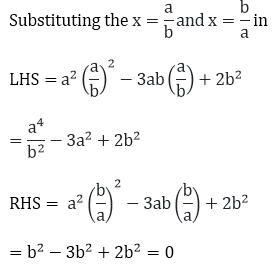≠ RHS

And, for x = b/a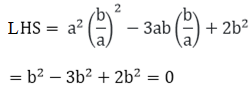= RHS

Therefore, x = b/a is a solution of the given equation.

### RD Sharma Solutions for Class 10 Maths Chapter 8 Exercise 8.2 Page No: 8.8

1. The product of two consecutive positive integers is 306. Form the quadratic equation to find the integers, if x denotes the smaller integer.

Solution:

Let the two integers be x and x+1, x taken as the smaller integer.

From the question, the product of these two integers is 306.

So,

x(x + 1) = 306

⇒ x+ x – 306 = 0

Thus, the required quadratic equation is x+ x – 306 = 0

2. John and Jivani together have 45 marbles. Both of them lost 5 marbles each, and the product of the number of marbles they now have is 128. Form the quadratic equation to find how many marbles they to start with, if John had x marbles.

Solution:

Given,

John and Jilani together have a total of 45 marbles.

Let John have x marbles.

So, Jivani will have (45 – x) marbles.

Number of marbles John had after losing 5 marbles = x – 5

Number of marbles Jivani had after losing 5 marbles = (45 – x) – 5 = 40 – x

Now, according to the question, the product of the marbles that they are having now is 128.

So,

(x – 5)(40 – x) = 128

⇒ 40x – x– 200 = 128

⇒ x2 – 45x + 128 + 200 = 0

⇒ x2 – 45x + 328 = 0

Thus the required quadratic equation is x2 – 45x + 328 = 0.

3. A cottage industry produces a certain number of toys in a day. The cost of production of each toy (in rupees) was found to be 55 minus the number of articles produced in a day. On a particular day, the total cost of production was Rs. 750. If x denotes the number of toys produced that day, form the quadratic equation to find x.

Solution:

Given that x denotes the number of toys produced in a day.

So, the cost of production of each toy = (55 – x)

And the total cost of production is the product of the number of toys produced in a day and the cost of production of each toy, i.e., x (55 – x)

From the question, it’s given that

The total cost of production on that particular day is Rs.750.

So,

⇒ x (55 – x) = 750

⇒ 55x – x2 = 750

⇒ x– 55x + 750 = 0

Thus, the required quadratic equation is x– 55x + 750 = 0

### RD Sharma Solutions for Class 10 Maths Chapter 8 Exercise 8.3 Page No: 8.19

Solve the following quadratic equation by factorisation:

1. (x – 4)(x + 2) = 0

Solution:

Given,

(x – 4) (x + 2) = 0

So, either x – 4 = 0 ⇒ x = 4

Or, x + 2 = 0, ⇒ x = – 2

Thus, the roots of the given quadratic equation are 4 and -2, respectively.

2. (2x + 3) (3x – 7) = 0

Solution:

Given,

(2x + 3) (3x – 7) = 0.

So, either 2x + 3 = 0, ⇒ x = – 3/2

Or, 3x -7 = 0, ⇒ x = 7/3

Thus, the roots of the given quadratic equation are x = -3/2 and x = 7/3, respectively.

3. 3x– 14x – 5 = 0

Solution:

Given.

3x– 14x – 5 = 0

⇒ 3x– 14x – 5 = 0

⇒ 3x– 15x + x – 5 = 0

⇒ 3x(x – 5) + 1(x – 5) = 0

⇒ (3x + 1)(x – 5) = 0

Now, either 3x + 1 = 0 ⇒ x = -1/3

Or, x – 5 = 0 ⇒ x = 5

Thus, the roots of the given quadratic equation are 5 and x = – 1/3, respectively.

4. Find the roots of equation 9x– 3x – 2 = 0.

Solution:

Given,

9x– 3x – 2 = 0.

⇒ 9x– 3x – 2 = 0.

⇒ 9x2 – 6x + 3x – 2 = 0

⇒ 3x (3x – 2) + 1(3x – 2) = 0

⇒ (3x – 2)(3x + 1) = 0

Now, either 3x – 2 = 0 ⇒ x = 2/3

Or, 3x + 1= 0 ⇒ x = -1/3

Thus, the roots of the given quadratic equation are x = 2/3 and x = -1/3, respectively.

5.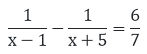Solution:

Given,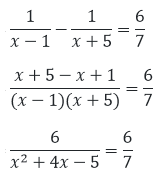Dividing by 6 both sides and cross-multiplying, we get

x2+ 4x – 12 = 0

⇒ x+ 6x – 2x – 12 = 0

⇒ x(x + 6) – 2(x – 6) = 0

⇒ (x + 6)(x – 2) = 0

Now, either x + 6 = 0 ⇒x = -6

Or, x – 2 = 0 ⇒ x = 2

Thus, the roots of the given quadratic equation are 2 and – 6, respectively.

6. 6x+ 11x + 3 = 0

Solution:

Given equation is 6x+ 11x + 3 = 0.

⇒ 6x+ 9x + 2x + 3 = 0

⇒ 3x (2x + 3) + 1(2x + 3) = 0

⇒ (2x +3) (3x + 1) = 0

Now, either 2x + 3 = 0 ⇒ x = -3/2

Or, 3x + 1= 0 ⇒ x = -1/3

Thus, the roots of the given quadratic equation are x = -3/2 and x = -1/3, respectively.

7. 5x– 3x – 2 = 0

Solution:

Given equation is 5x– 3x – 2 = 0.

⇒ 5x– 3x – 2 = 0.

⇒ 5x2 – 5x + 2x – 2 = 0

⇒ 5x(x – 1) + 2(x – 1) = 0

⇒ (5x + 2)(x – 1) = 0

Now, either 5x + 2 = 0 ⇒x = -2/5

Or, x -1= 0 ⇒x = 1

Thus, the roots of the given quadratic equation are x = 1 and x = -2/5, respectively.

8. 48x– 13x – 1 = 0

Solution:

Given equation is 48x– 13x – 1 = 0.

⇒ 48x– 13x – 1 = 0.

⇒ 48x– 16x + 3x – 1 = 0.

⇒ 16x(3x – 1) + 1(3x – 1) = 0

⇒ (16x + 1)(3x – 1) = 0

Either 16x + 1 = 0 ⇒ x = -1/16

Or, 3x – 1=0 ⇒ x = 1/3

Thus, the roots of the given quadratic equation are x = -1/16 and x = 1/3, respectively.

9. 3x= -11x – 10

Solution:

Given equation is 3x= -11x – 10

⇒ 3x+ 11x + 10 = 0

⇒ 3x+ 6x + 5x + 10 = 0

⇒ 3x(x + 2) + 5(x + 2) = 0

⇒ (3x + 5)(x + 2) = 0

Now, either 3x + 5 = 0 ⇒ x = -5/3

Or, x + 2 = 0 ⇒ x = -2

Thus, the roots of the given quadratic equation are x = -5/3 and -2, respectively.

10. 25x(x + 1) = – 4

Solution:

Given equation is 25x(x + 1) = -4

25x(x + 1) = -4

⇒ 25x+ 25x + 4 = 0

⇒ 25x+ 20x + 5x + 4 = 0

⇒ 5x (5x + 4) + 1(5x + 4) = 0

⇒ (5x + 4)(5x + 1) = 0

Now, either 5x + 4 = 0 therefore x = – 4/5

Or, 5x + 1 = 0 therefore x = -1 /5

Thus, the roots of the given quadratic equation are x = – 4/5 and x = -1/5, respectively.

11. 16x – 10/x = 27

Solution:

Given,

16x – 10/x = 27

On multiplying x on both sides, we have,

⇒ 16x2 – 10 = 27x

⇒ 16x2 – 27x – 10 = 0

⇒ 16x2 – 32x + 5x – 10 = 0

⇒ 16x(x – 2) +5(x – 2) = 0

⇒ (16x + 5) (x – 2) = 0

Now, either 16x + 5 = 0 ⇒ x = -5/16

Or, x – 2 = 0 ⇒ x = 2

Thus, the roots of the given quadratic equation are x = – 5/16 and x = 2, respectively.

12.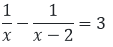Solution:

The given equation is,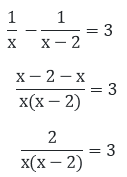On cross multiplying on both sides, we get,

2 = 3x(x – 2)

2 = 3x– 6x

3x2– 6x – 2 = 0

⇒ 3x2– 3x – 3x – 2 = 0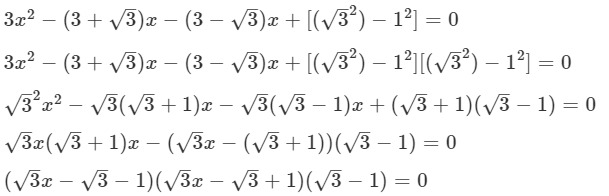Now, either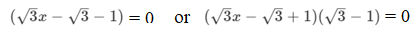Thus,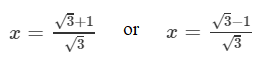are the solutions of the given quadratic equations.

13. x – 1/x = 3, x ≠ 0

Solution:

Given,

x – 1/x = 3

On multiplying x on both sides, we have,

⇒ x2 – 1 = 3x

⇒ x2 – 3x – 1 = 0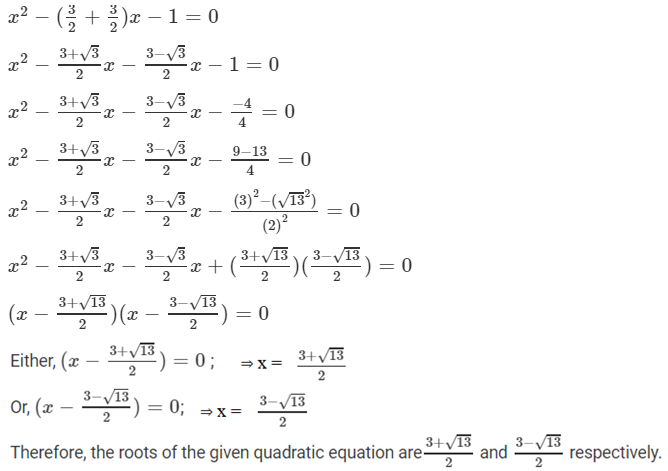14.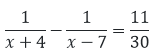Solution:

Given,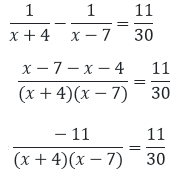Dividing by 11 both the sides and cross-multiplying, we get,

⇒ x– 3x – 28 = – 30

⇒ x– 3x – 2 = 0

⇒ x– 2x – x – 2 = 0

⇒ x(x – 2) – 1(x – 2) = 0

⇒ (x – 2)(x – 1) = 0

Now, either x – 2 = 0 ⇒ x = 2

Or, x – 1 = 0 ⇒ x = 1

Thus, the roots of the given quadratic equation are 1 and 2, respectively.

15.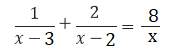Solution:

Given,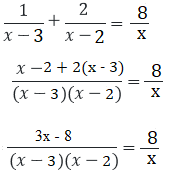On cross multiplying, we get,

⇒ x(3x – 8) = 8(x – 3)(x – 2)

⇒ 3x2 – 8x = 8(x2 – 5x + 6)

⇒ 8x2 – 40x + 48 – (3x2 – 8x) = 0

⇒ 5x2 – 32x + 48 = 0

⇒ 5x2 – 20x – 12x + 48 = 0

⇒ 5x(x – 4) – 12(x – 4) = 0

⇒ (x – 4)(5x – 12) = 0

Now, either x – 4 = 0 ⇒ x = 4

Or, 5x – 12 = 0 ⇒ x = 12/5

Thus, the roots of the given quadratic equation are 12/5 and 4, respectively.

16. a2x– 3abx + 2b= 0

Solution:

Given equation is a2x– 3abx + 2b= 0

⇒ a2x– abx – 2abx + 2b= 0

⇒ ax(ax – b) – 2b(ax – b) = 0

⇒ (ax – b)(ax – 2b) = 0

Now, either ax – b = 0 ⇒ x = b/a

Or, ax – 2b = 0 ⇒ x = 2b/a

Thus, the roots of the quadratic equation are x = 2b/a and x = b/a, respectively.

17. 9x2 – 6b2x – (a4 – b4) = 0

Solution:

Given,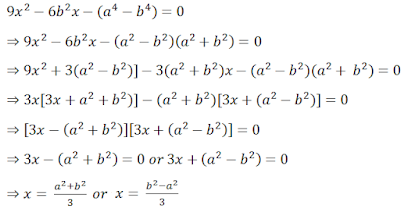Thus, the roots of the quadratic equation are x = (b2 – a2)/3 and x = (a2 + b2)/3, respectively.

18. 4x+ 4bx – (a– b2) = 0

Solution:

Given,

4x+ 4bx – (a– b2) = 0

For factorizing,

4(a– b2) = -4(a – b) (a + b) = [-2(a-b)] [2(a + b)]

⇒ 2(b – a)*2(b + a)

⇒ 4x2+ (2(b – a) + 2(b + a)) – (a – b)(a + b) = 0

So, now

4x2  + 2(b – a)x++ 2(b + a)x + (b – a)(a + b) = 0

⇒ 2x(2x + (b – a)) +(a + b)(2x + (b – a)) = 0

⇒ (2x + (b – a))(2x + b + a) = 0

Now, either (2x + (b – a)) = 0 ⇒x = (a – b)/2

Or, (2x + b + a) = 0 ⇒ x = -(a + b)/2

Thus, the roots of the given quadratic equation are x = -(a + b)/2 and x = (a – b)/2, respectively.

19. ax+ (4a– 3b)x – 12ab = 0

Solution:

Given equation is ax+ (4a– 3b)x – 12ab = 0

⇒ ax+ 4a2x – 3bx – 12ab = 0

⇒ ax(x + 4a) – 3b(x + 4a) = 0

⇒ (x + 4a)(ax – 3b) = 0

Now, either x + 4a = 0 ⇒ x = -4a

Or, ax – 3b = 0 ⇒ x = 3b/a

Thus, the roots of the given quadratic equation are x = 3b/a and -4a, respectively.

20. 2x2 + ax – a2 = 0

Solution:

Given,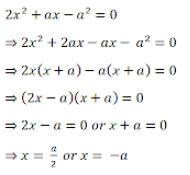Thus, the roots of the given quadratic equation are x = a/2 and -a, respectively.

21. 16/x – 1 = 15/(x + 1), x ≠ 0, -1

Solution:

Given,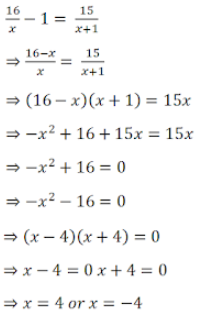Thus, the roots of the given quadratic equation are x = 4 and -4, respectively.

22.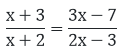, x ≠ -2, 3/2

Solution:

Given,On cross-multiplying, we get,

(x + 3)(2x – 3) = (x + 2)(3x – 7)

⇒ 2x– 3x + 6x – 9 = 3x– x – 14

⇒ 2x+ 3x – 9 = 3x– x – 14

⇒ x– 3x – x – 14 + 9 = 0

⇒ x– 5x + x – 5 = 0

⇒ x(x – 5) + 1(x – 5) = 0

⇒ (x – 5)(x + l) – 0

Now, either x – 5 = 0 or x + 1 = 0

⇒ x = 5 and x = -1

Thus, the roots of the given quadratic equation are 5 and -1, respectively.

23.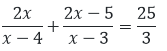, x ≠ 3, 4

Solution:

The given equation is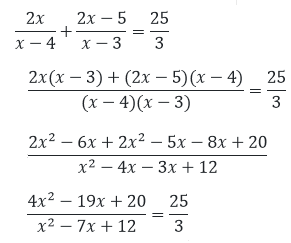On cross multiplying, we have

3(4x– 19x + 20) = 25(x– 7x + 12)

⇒ 12x– 57x + 60 = 25x2 – 175x + 300

⇒13x– 78x – 40x + 240 = 0

⇒13x– 118x + 240 = 0

⇒13x– 78x – 40x + 240 = 0

⇒13x(x – 6) – 40(x – 6) = 0

⇒ (x – 6)(13x – 40) = 0

Now, either x – 6 = 0 ⇒x = 6

Or, 13x – 40 = 0 ⇒x = 40/13

Thus, the roots of the given quadratic equation are 6 and 40/13, respectively.

24.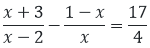x ≠ 0, 2

Solution:

The given equation is,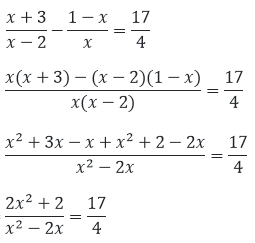On cross multiplying, we get

4(2x+ 2) = 17(x– 2x)

⇒ 8x+ 8 = 17x– 34x

⇒ 9x– 34x – 8 = 0

⇒ 9x– 36x + 2x – 8 = 0

⇒ 9x(x – 4) + 2(x – 4) = 0

⇒ 9x + 2)(x – 4) = 0

Now, either 9x + 2 = 0 ⇒x = -2/9

Or, x – 4 = 0 ⇒ x = 4

Thus, the roots of the given quadratic equation are x = -2/9 and 4, respectively.

25.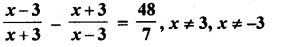Solution:

The given equation is,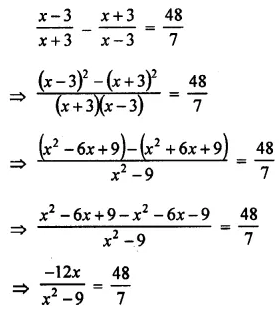On cross-multiplying, we get

7(-12x) = 48(x2 – 9)

⇒ -84x = 48x2 – 432

⇒ 48x2 + 84x – 432 = 0

⇒ 4x2 + 7x – 36 = 0 dividing by 12]

⇒ 4x2 + 16x – 9x – 36 = 0

⇒ 4x(x + 4) – 9(x – 4) = 0

⇒ (4x – 9)(x + 4) = 0

Now, either 4x – 9 = 0 ⇒x = 9/4

Or, x + 4 = 0 ⇒ x = -4

Thus, the roots of the given quadratic equation are x = 9/4 and -4, respectively.

26.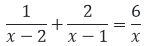, x ≠ 0

Solution:

The given equation is,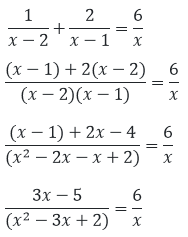On cross multiplying, we have

x(3x – 5) = 6(x– 3x + 2)

⇒ 3x– 5x = 6x– 18x + 12

⇒ 3x– 13x + 12 = 0

⇒ 3x– 9x – 4x + 12 = 0

⇒ 3x(x – 3) – 4(x – 3) = 0

⇒ (x – 3)(3x – 4) = 0

Now, either x – 3 = 0 ⇒ x = 3

Or, 3x – 4 = 0 ⇒ 4/3.

Thus, the roots of the given quadratic equation are 3 and 4/3, respectively.

27.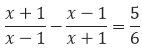, x ≠ 1, -1

Solution:

The given equation is,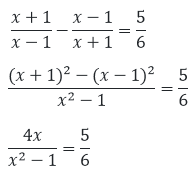On cross-multiplying, we have,

⇒ 6(4x) = 5(x– 1) = 24x

⇒ 5x– 5 = 5x– 24x – 5 =0

⇒ 5x– 25x + x – 5 = 0

⇒ 5x(x – 5) + 1(x – 5) = 0

⇒ (5x + 1)(x – 5) = 0

Now, either x – 5 = 0 ⇒ x = 5

Or, 5x + 1 = 0 ⇒ x = −1/5

Thus, the roots of the given quadratic equation are x = −1/5 and 5, respectively.

28.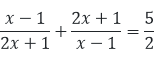, x ≠ 1, -1/2

Solution:

The given equation is,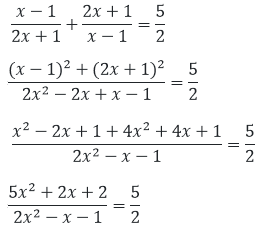On cross-multiplying, we have,

⇒ 2(5x+ 2x + 2) = 5(2x– x – 1)

⇒ 10x+ 4x + 4 = 10x– 5x – 5

⇒ 4x + 5x + 4 + 5 = 0

⇒ 9x + 9 = 0

⇒ 9x = -9

Thus, x = -1 is the only root of the given equation.

29.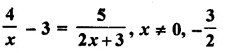Solution:

The given equation is,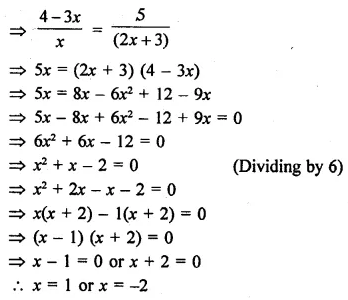Thus, the roots of the given quadratic equation are x = 1 and x = -2, respectively.

30.

$$\begin{array}{l}\frac{x-4}{x-5}+\frac{x-6}{x-7}=\frac{10}{3},x\neq 5, 7\end{array}$$

Solution:

The given equation is,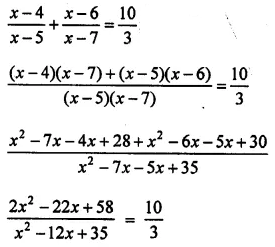On cross-multiplying, we have,

3(2x2 – 22x + 58) = 10(x2 – 12x + 35)

⇒ 6x2 – 66x + 174 = 10x2 – 120x + 350

⇒ 4x2 – 54x + 176 = 0

⇒ 2x2 – 27x + 88 = 0

⇒ 2x2 – 16x – 11x + 88 = 0

⇒ 2x(x – 8) – 11(x + 8) = 0

⇒ (x – 8)(2x – 11) = 0

Now, either x – 8 = 0 ⇒ x = 8

Or, 2x – 11 = 0 ⇒ x = 11/2

Thus, the roots of the given quadratic equation are x = 11/2 and 8, respectively.

### RD Sharma Solutions for Class 10 Maths Chapter 8 Exercise 8.4 Page No: 8.26

Find the roots of the following quadratic equations (if they exist) by the method of completing the square.

1. x2 – 4√2 x + 6 = 0

Solution:

Given equation,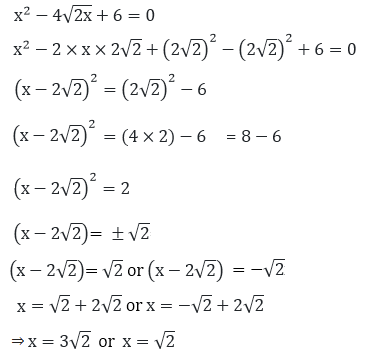Thus, the roots of the given quadratic equation are x = 3√2 and x = √2.

2. 2x2 – 7x + 3 = 0

Solution:

Given equation,

2x2 – 7x + 3 = 0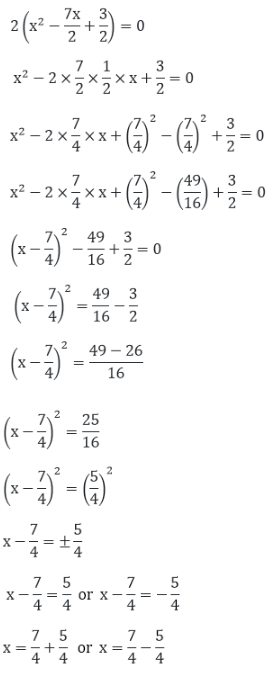⇒ x = 12/4 = 3 or x = 2/4 = 1/2

Thus, the roots of the given quadratic equation are x = 3 and x = 1/2.

3. 3x2 + 11x + 10 = 0

Solution:

Given equation,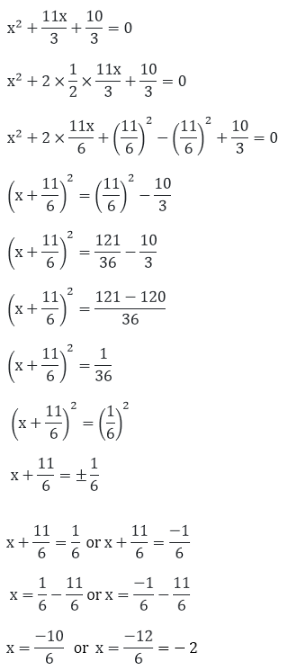⇒ x = -5/3 = 3 or x = -2

Thus, the roots of the given quadratic equation are x = -5/3 and x = -2.

4. 2x2 + x – 4 = 0

Solution:

Given equation,

2x2 + x – 4 = 0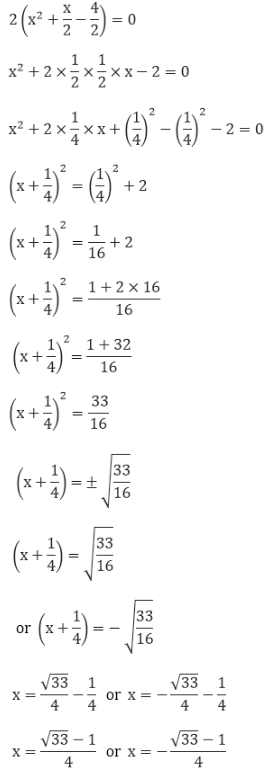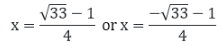Thus, the roots of the given quadratic equation are

$$\begin{array}{l}x = \frac{\sqrt{33}-1}{4} or x = – \frac{\sqrt{33}-1}{4}\end{array}$$

5. 2x2 + x + 4 = 0

Solution:

Given equation,

2x2 + x + 4 = 0

x2 + x/2 + 2 = 0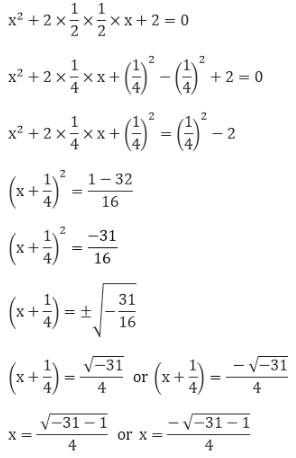Thus, the above are the two roots of the given quadratic equation.

### RD Sharma Solutions for Class 10 Maths Chapter 8 Exercise 8.5 Page No: 8.32

1. Write the discriminant of the following quadratic equations:

(i) 2x2 – 5x + 3 = 0

Solution:

Given equation,

2x2 – 5x + 3 = 0

It is in the form of ax2 + bx + c = 0

Where, a = 2, b = -5 and c = 3

So, the discriminant is given by D = b2 – 4ac

D = (-5)2 – 4 x 2 x 3

D = 25 – 24 = 1

Hence, the discriminant of the given quadratic equation is 1.

(ii) x2 + 2x + 4 = 0

Solution:

Given equation,

x2 + 2x + 4 = 0

It is in the form of ax2 + bx + c = 0

Where, a = 1, b = 2 and c = 4

So, the discriminant is given by D = b2 – 4ac

D = (2)2 – 4 x 1 x 4

D = 4 – 16 = – 12

Hence, the discriminant of the given quadratic equation is – 12.

(iii) (x – 1)(2x – 1) = 0

Solution:

Given equation,

(x -1) (2x -1) = 0

On expanding it, we get

2x2 – 3x + 1 = 0

It is in the form of ax2 + bx + c = 0

Where, a = 2, b = -3, c = 1

So, the discriminant is given by D = b2 – 4ac

D = (-3)2 – 4 x 2 x 1

D = 9 – 8 = 1

Hence, the discriminant of the given quadratic equation is 1.

(iv) x2 -2x + k = 0, k ∈ R

Solution:

Given equation,

x– 2x + k = 0

It is in the form of ax2 + bx + c = 0

Where, a = 1, b = -2, and c = k

So, the discriminant is given by D = b2 – 4ac

D = (-2)2 – 4(1)(k)

= 4 – 4k

Hence, the discriminant of the given equation is (4 – 4k).

(v)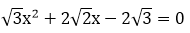Solution: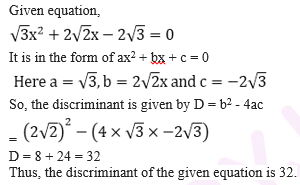(vi) x2 – x + 1 = 0

Solution:

Given equation,

x2 – x + 1 = 0 It is in the form of ax2 + bx + c = 0

Where, a = 1, b = -1 and c = 1

So, the discriminant is given by D = b2 – 4ac

D = (-1)2 – 4 × 1 × 1

D = 1 – 4 = – 3

Thus, the discriminant of the given equation is -3.

### RD Sharma Solutions for Class 10 Maths Chapter 8 Exercise 8.6 Page No: 8.41

1. Determine the nature of the roots of the following quadratic equations:

Important Notes:

– A quadratic equation is in the form ax+bx +c =0

– To find the nature of roots, first, find determinant “D”.

– D = b2 – 4ac

– If D > 0, the equation has real and distinct roots.

– If D < 0, the equation has no real roots.

– If D = 0, the equation has 1 root.

(i) 2x2 -3x + 5 =0

Solution:

Here, a= 2, b= -3, c= 5

D = b2 – 4ac

= (-3)2 -4(2)(5)

= 9 – 40

= -31<0

It’s seen that D<0, and hence, the given equation does not have any real roots.

(ii) 2x2 -6x + 3=0

Solution:

Here, a= 2, b= -6, c= 3

D = (-6)2 -4(2)(3)

= 36 – 24

= 12>0

It’s seen that D>0, and hence, the given equation have real and distinct roots.

(iii) (3/5)x2 – (2/3) + 1 = 0

Solution:

Here, a= 3/5, b= -2/3, c= 1

D = (-2/3)2 -4(3/5)(1)

= 4/9 – 12/5

= -88/45<0

It’s seen that D<0, and hence, the given equation does not have any real roots.

(iv) 3x2 – 4√3x + 4 = 0

Solution:

Here, a= 3, b= – 43, c= 4

D = (- 43)2 -4(3)(4)

= 48 – 48

= 0

It’s seen that D = 0, and hence, the given equation has only 1 real and equal root.

(v) 3x2 – 2√6x + 2 = 0

Solution:

Here, a= 3, b= – 26, c= 2

D = (- 26)2 – 4(3)(2)

= 24 – 24

= 0

It’s seen that D = 0, and hence, the given equation has only 1 real and equal root.

2. Find the values of k for which the roots are real and equal in each of the following equations:

(i) kx+ 4x + 1 = 0

Solution:

The given equation kx+ 4x + 1 = 0 is in the form of ax+ bx + c = 0

Where a = k, b = 4, c = 1

For the equation to have real and equal roots, the condition is

D = b– 4ac = 0

⇒ 4– 4(k)(1) = 0

⇒ 16 – 4k = 0

⇒ k = 4

The value of k is 4.

(ii) kx2 – 2√5x + 4 = 0

Solution:

The given equation kx– 2√5x + 4 = 0 is in the form of ax+ bx + c = 0

Where a = k, b = – 2√5, c = 4

For the equation to have real and equal roots, the condition is

D = b– 4ac = 0

⇒ (- 2√5)– 4(k)(4) = 0

⇒ 20 –16k = 0

⇒ k = 5/4

The value of k is 5/4.

(iii) 3x– 5x + 2k = 0

Solution:

The given equation 3x– 5x + 2k = 0 is in the form of ax+ bx + c = 0

Where a = 3, b = -5, c = 2k

For the equation to have real and equal roots, the condition is

D = b– 4ac = 0

⇒ (-5)– 4(3)(2k) = 0

⇒ 25 – 24k = 0

⇒ k = 25/24

The value of k is 25/24.

(iv) 4x+ kx + 9 = 0

Solution:

The given equation 4x+ kx + 9 = 0 is in the form of ax+ bx + c = 0

Where a = 4, b = k, c = 9

For the equation to have real and equal roots, the condition is

D = b– 4ac = 0

⇒ k– 4(4)(9) = 0

⇒ k2 – 144 = 0

⇒ k = ± 12

The value of k is 12 or -12.

(v) 2kx– 40x + 25 = 0

Solution:

The given equation 2kx– 40x + 25 = 0 is in the form of ax+ bx + c = 0

Where a = 2k, b = -40, c = 25

For the equation to have real and equal roots, the condition is

D = b– 4ac = 0

⇒ (-40)– 4(2k)(25) = 0

⇒ 1600 – 200k = 0

⇒ k = 8

The value of k is 8.

(vi) 9x– 24x + k = 0

Solution:

The given equation 9x– 24x + k = 0 is in the form of ax+ bx + c = 0

Where a = 9, b = -24, c = k

For the equation to have real and equal roots, the condition is

D = b– 4ac = 0

⇒ (-24)– 4(9)(k) = 0

⇒ 576 – 36k = 0

⇒ k = 16

The value of k is 16.

(vii) 4x2– 3kx + 1 = 0

Solution:

The given equation 4x– 3kx + 1 = 0 is in the form of ax+ bx + c = 0

Where a = 4, b = -3k, c = 1

For the equation to have real and equal roots, the condition is

D = b– 4ac = 0

⇒ (-3k)– 4(4)(1) = 0

⇒ 9k2 – 16 = 0

⇒ k = ± 4/3

The value of k is ± 4/3.

(viii) x– 2(5 + 2k)x + 3(7 + 10k) = 0

Solution:

The given equation x– 2(5 + 2k)x + 3(7 + 10k) = 0 is in the form of ax+ bx + c = 0

Where a = 1, b = -2(5 + 2k), c = 3(7 + 10k)

For the equation to have real and equal roots, the condition is

D = b– 4ac = 0

⇒ (-2(5 + 2k))– 4(1)( 3(7 + 10k)) = 0

⇒ 4(5 + 2k)– 12(7 + 10k) = 0

⇒ 25 + 4k+ 20k – 21 – 30k = 0

⇒ 4k– 10k + 4 = 0

⇒ 2k– 5k + 2 = 0 [dividing by 2]

Now, solving for k by factorisation, we have

⇒ 2k– 4k – k + 2 = 0

⇒ 2k(k – 2) – 1(k – 2) = 0

⇒ (k – 2)(2k – 1) = 0,

k = 2 and k = 1/2,

So, the value of k can either be 2 or 1/2.

(ix) (3k +1)x2+ 2(k +1)x + k = 0

Solution:

The given equation (3k +1)x+ 2(k +1)x + k = 0 is in the form of ax+ bx + c = 0

Where a = (3k +1), b = 2(k + 1), c = k

For the equation to have real and equal roots, the condition is

D = b– 4ac = 0

⇒ (2(k + 1))– 4(3k +1)(k) = 0

⇒ 4(k +1)– 4(3k2 + k) = 0

⇒ (k + 1)– k(3k + 1) = 0

⇒ 2k– k – 1 = 0

Now, solving for k by factorisation, we have

⇒ 2k– 2k + k – 1 = 0

⇒ 2k(k – 1) + 1(k – 1) = 0

⇒ (k – 1)(2k + 1) = 0,

k = 1 and k = -1/2,

So, the value of k can either be 1 or -1/2.

(x) kx+ kx + 1 = – 4x– x

Solution:

The given equation kx+ kx + 1 = -4x2 – x

This can be rewritten as,

(k + 4)x2 + (k + 1)x + 1 = 0

Now, this is in the form of ax+ bx + c = 0

Where a = (k +4), b = (k + 1), c = 1

For the equation to have real and equal roots, the condition is

D = b– 4ac = 0

⇒ (k + 1)– 4(k +4)(1) = 0

⇒ (k +1)– 4k – 16 = 0

⇒ k2 + 2k + 1 – 4k – 16 = 0

⇒ k– 2k – 15 = 0

Now, solving for k by factorisation, we have

⇒ k– 5k + 3k – 15 = 0

⇒ k(k – 5) + 3(k – 5) = 0

⇒ (k + 3)(k – 5) = 0,

k = -3 and k = 5,

So, the value of k can either be -3 or 5.

(xi) (k + 1)x+ 2(k + 3)x + (k + 8) = 0

Solution:

The given equation (k +1)x+ 2(k +3)x + (k +8) = 0 is in the form of ax+ bx + c = 0

Where a = (k +1), b = 2(k + 3), c = (k + 8)

For the equation to have real and equal roots, the condition is

D = b– 4ac = 0

⇒ (2(k + 3))– 4(k +1)(k + 8) = 0

⇒ 4(k +3)– 4(k2 + 9k + 8) = 0

⇒ (k + 3)– (k2 + 9k + 8) = 0

⇒ k+6k + 9 – k2 – 9k – 8 = 0

⇒ -3k + 1 = 0

⇒ k = 1/3

So, the value of k is 1/3.

(xii) x– 2kx + 7k – 12 = 0

Solution:

The given equation x– 2kx + 7k – 12 = 0 is in the form of ax+ bx + c = 0

Where a = 1, b = -2k, c = 7k – 12

For the equation to have real and equal roots, the condition is

D = b– 4ac = 0

⇒ (-2k)– 4(1)(7k – 12) = 0

⇒ 4k– 4(7k – 12) = 0

⇒ k2 – 7k + 12 = 0

Now, solving for k by factorisation, we have

⇒ k– 4k – 3k + 12 = 0

⇒ (k – 4)(k – 3) = 0,

k = 4 and k = 3,

So, the value of k can either be 4 or 3.

(xiii) (k + 1)x– 2(3k + 1)x + 8k + 1 = 0

Solution:

The given equation (k +1)x– 2(3k +1)x + 8k + 1 = 0 is in the form of ax+ bx + c = 0

Where a = (k +1), b = -2(3k + 1), c = 8k + 1

For the equation to have real and equal roots, the condition is

D = b– 4ac = 0

⇒ (-2(3k + 1))– 4(k +1)(8k + 1) = 0

⇒ 4(3k +1)– 4(k + 1)(8k + 1) = 0

⇒ (3k + 1)– (k + 1)(8k + 1) = 0

⇒ 9k+ 6k + 1 – (8k+ 9k + 1) = 0

⇒ 9k+ 6k + 1 – 8k– 9k – 1 = 0

⇒ k– 3k = 0

⇒ k(k – 3) = 0

Either k = 0 Or, k – 3 = 0 ⇒ k = 3,

So, the value of k can either be 0 or 3

(xiv) 5x– 4x + 2 + k(4x– 2x + 1) = 0

Solution:

The given equation 5x– 4x + 2 + k(4x– 2x + 1) = 0

This can be rewritten as,

x2(5 + 4k) – x(4 + 2k) + 2 – k = 0

Now, this is in the form of ax+ bx + c = 0

Where a = (4k +5), b = -(2k + 4), c = 2 – k

For the equation to have real and equal roots, the condition is

D = b– 4ac = 0

⇒ (-(2k + 4))– 4(4k +5)(2 – k) = 0

⇒ (2k +4)– 4(4k +5)(2 – k) = 0

⇒ 16 + 4k+ 16k – 4(10 – 5k + 8k – 4k2) = 0

⇒ 16 + 4k+ 16k – 40 + 20k – 32k + 16k2 = 0

⇒ 20k+ 4k – 24 = 0

⇒ 5k2 + k – 6 = 0

Now, solving for k by factorisation, we have

⇒ 5k+ 6k – 5k – 6 = 0

⇒ 5k(k – 1) + 6(k – 1) = 0

⇒ (k – 1)(5k + 6) = 0,

k = 1 and k = -6/5,

So, the value of k can either be 1 or -6/5.

(xv) (4 – k)x+ (2k + 4)x + (8k + 1) = 0

Solution:

The given equation (4 – k)x+ (2k + 4)x + (8k + 1) = 0 is in the form of ax+ bx + c = 0

Where a = (4 – k), b = (2k + 4), c = (8k + 1)

For the equation to have real and equal roots, the condition is

D = b– 4ac = 0

⇒ (2k + 4)– 4(4 – k)(8k + 1) = 0

⇒ 4k+ 16k + 16 – 4(-8k+ 32k + 4 – k) = 0

⇒ 4k+ 16k + 16 + 32k– 124k – 16 = 0

⇒ 36k– 108k = 0

Taking common,

⇒ 9k(k -3 ) = 0

Now, either 9k = 0 ⇒ k = 0 or k – 3 = 0 ⇒ k = 3,

So, the value of k can either be 0 or 3.

(xvi) (2k + 1)x+ 2(k + 3)x + (k +5) = 0

Solution:

The given equation (2k +1)x+ 2(k +3)x + (k + 5) = 0 is in the form of ax+ bx + c = 0

Where a = (2k +1), b = 2(k + 3), c = (k + 5)

For the equation to have real and equal roots, the condition is

D = b– 4ac = 0

⇒ (2(k + 3))– 4(2k +1)(k + 5) = 0

⇒ 4(k +3)– 4(2k2 + 11k + 5) = 0

⇒ (k + 3)– (2k2 + 11k + 5) = 0 [dividing by 4 both sides]

⇒ k+ 5k – 4 = 0

Now, solving for k by completing the square, we have

⇒ k+ 2 x (5/2) x k + (5/2)2 = 4 + (5/2)2

⇒ (k + 5/2)2 = 4 + 25/4 = 41/4

⇒ k + (5/2) = ± 41/2

⇒ k = (41 – 5)/2 or –(41 + 5)/2

So, the value of k can either be (41 – 5)/2 or –(41 + 5)/2

(xvii) 4x– 2(k + 1)x + (k + 4) = 0

Solution:

The given equation 4x– 2(k +1)x + (k + 4)= 0 is in the form of ax+ bx + c = 0

Where a = 4, b = -2(k + 1), c = (k + 4)

For the equation to have real and equal roots, the condition is

D = b– 4ac = 0

⇒ (-2(k + 1))– 4(4)(k + 4) = 0

⇒ 4(k +1)– 16(k + 4) = 0

⇒ (k + 1)– 4(k + 4) = 0

⇒ k– 2k – 15 = 0

Now, solving for k by factorisation, we have

⇒ k– 5k + 3k – 15 = 0

⇒ k(k – 5) + 3(k – 5) = 0

⇒ (k – 5)(k + 3) = 0,

k = 5 and k = -3,

So, the value of k can either be 5 or -3.

3. In the following, determine the set of values of k for which the given quadratic equation has real roots:

(i) 2x+ 3x + k = 0

Solution:

Given,

2x+ 3x + k = 0

It’s of the form of ax+ bx + c = 0

Where, a = 2, b = 3, c = k

For the given quadratic equation to have real roots D = b2– 4ac ≥ 0

D = 9 – 4(2)(k) ≥ 0

⇒ 9 – 8k ≥ 0

⇒ k ≤ 9/8

The value of k should not exceed 9/8 to have real roots.

(ii) 2x+ x + k = 0

Solution:

Given,

2x+ x + k = 0

It’s of the form of ax+ bx + c = 0

Where, a = 2, b = 1, c = k

For the given quadratic equation to have real roots D = b2 – 4ac ≥ 0

D = 12 – 4(2)(k) ≥ 0

⇒ 1 – 8k ≥ 0

⇒ k ≤ 1/8

The value of k should not exceed 1/8 to have real roots.

(iii) 2x– 5x – k = 0

Solution:

Given,

2x– 5x – k = 0

It’s of the form of ax+ bx + c = 0

Where, a = 2, b = -5, c = -k

For the given quadratic equation to have real roots D = b2 – 4ac ≥ 0

D = (-5)2 – 4(2)(-k) ≥ 0

⇒ 25 + 8k ≥ 0

⇒ k ≥ -25/8

The value of k should be lesser than -25/8 to have real roots.

(iv) kx+ 6x + 1 = 0

Solution:

Given,

kx+ 6x + 1 = 0

It’s of the form of ax+ bx + c = 0

Where, a = k, b = 6, c = 1

For the given quadratic equation to have real roots D = b2 – 4ac ≥ 0

D = 62 – 4(k)(1) ≥ 0

⇒ 36 – 4k ≥ 0

The given equation will have real roots if,

36 – 4k ≥ 0

36 ≥ 4k

36/4 ≥ k

9 ≥ k

⇒ so, k ≤ 9

The value of k should not exceed 9 to have real roots.

(v) 3x+ 2x + k = 0

Solution:

Given,

3x+ 2x + k = 0

It’s of the form of ax+ bx + c = 0

Where, a =3, b = 2, c = k

For the given quadratic equation to have real roots D = b2 – 4ac ≥ 0

D = (2)2 – 4(3)(k) ≥ 0

⇒ 4 – 12k ≥ 0

⇒ 4 ≥ 12k

⇒ k ≤ 1/3

The value of k should not exceed 1/3 to have real roots.

4. Find the values of k for which the following equations have real and equal roots

(i) x2 – 2(k + 1)x + k2 = 0

Solution:

Given,

x2 – 2(k + 1)x + k2 = 0

It’s of the form of ax+ bx + c = 0

Where, a =1, b = -2(k + 1), c = k2

For the given quadratic equation to have real roots D = b2 – 4ac = 0

D = (-2(k + 1))2 – 4(1)(k2) = 0

⇒ 4k2 + 8k + 4 – 4k2 = 0

⇒ 8k + 4 = 0

⇒ k = -4/8

⇒ k = -1/2

The value of k should -1/2 to have real and equal roots.

(ii)k2x2 – 2 (2k – 1)x + 4 = 0

Solution:

Given,

k2x2 – 2 (2k – 1)x + 4 = 0

It’s of the form of ax+ bx + c = 0

Where, a = k2, b = –2 (2k – 1), c = 4

For the given quadratic equation to have real roots D = b2 – 4ac = 0

D = (-2(2k – 1))2 – 4(4)(k2) = 0

⇒ 4k2 – 4k + 1 – 4k2 = 0 [dividing by 4 both sides]

⇒ -4k + 1 = 0

⇒ k = 1/4

The value of k should 1/4 to have real and equal roots.

(iii) (k + 1)x2 – 2(k – 1)x + 1 = 0

Solution:

Given,

(k + 1)x2 – 2(k – 1)x + 1 = 0

It’s of the form of ax+ bx + c = 0

Where, a = (k + 1), b = -2(k – 1), c = 1

For the given quadratic equation to have real roots D = b2 – 4ac = 0

D = (-2(k – 1))2 – 4(1)(k + 1) = 0

⇒ 4k2 – 2k + 1 – k – 1 = 0 [dividing by 4 both sides]

⇒ k2 – 3k = 0

⇒ k(k – 3) = 0

⇒ k = 0 or k = 3

The value of k can be 0 or 3 to have real and equal roots.

5. Find the values of k for which the following equations have real roots

(i) 2x2 + kx + 3 = 0

Solution:

Given,

2x+ kx + 3 = 0

It’s of the form of ax+ bx + c = 0

Where, a = 2, b = k, c = 3

For the given quadratic equation to have real roots D = b2 – 4ac ≥ 0

D = (k)2 – 4(3)(2) ≥ 0

⇒ k2 – 24 ≥ 0

⇒ k2 ≥ 24

⇒ k ≥ 26 and k ≤ -26 [After taking square root on both sides]

The value of k can be represented as (∞, 26] U [-26, -∞)

(ii) kx(x – 2) + 6 = 0

Solution:

Given,

kx(x – 2) + 6 = 0

It can be rewritten as,

kx2 – 2kx + 6 = 0

It’s of the form of ax+ bx + c = 0

Where, a =k, b = -2k, c = 6

For the given quadratic equation to have real roots D = b2 – 4ac ≥ 0

D = (-2k)2 – 4(k)(6) ≥ 0

⇒ 4k2 – 24k ≥ 0

⇒ 4k(k – 6) ≥ 0

⇒ k ≥ 0 and k ≥ 6

⇒ k ≥ 6

The value of k should be greater than or equal to 6 to have real roots.

(iii) x2 – 4kx + k = 0

Solution:

Given,

x2 – 4kx + k = 0

It’s of the form of ax+ bx + c = 0

Where, a =1, b = -4k, c = k

For the given quadratic equation to have real roots D = b2 – 4ac ≥ 0

D = (-4k)2 – 4(1)(k) ≥ 0

⇒ 16k2 – 4k ≥ 0

⇒ 4k(4k – 1) ≥ 0

⇒ k ≥ 0 and k ≥ 1/4

⇒ k ≥ 1/4

The value of k should be greater than or equal to 1/4 to have real roots.

(iv) kx(x – 2√5) + 10 = 0

Solution:

Given,

kx(x – 2√5) + 10 = 0

It can be rewritten as,

kx2 – 2√5kx + 10 = 0

It’s of the form of ax+ bx + c = 0

Where, a =k, b = -2√5k, c = 10

For the given quadratic equation to have real roots D = b2 – 4ac ≥ 0

D = (-2√5k)2 – 4(k)(10) ≥ 0

⇒ 20k2 – 40k ≥ 0

⇒ 20k(k – 2) ≥ 0

⇒ k ≥ 0 and k ≥ 2

⇒ k ≥ 2

The value of k should be greater than or equal to 2 to have real roots.

(v) kx(x – 3) + 9 = 0

Solution:

Given,

kx(x – 3) + 9 = 0

It can be rewritten as,

kx2 – 3kx + 9 = 0

It’s of the form of ax+ bx + c = 0

Where, a = k, b = -3k, c = 9

For the given quadratic equation to have real roots D = b2 – 4ac ≥ 0

D = (-3k)2 – 4(k)(9) ≥ 0

⇒ 9k2 – 36k ≥ 0

⇒ 9k(k – 4) ≥ 0

⇒ k ≥ 0 and k ≥ 4

⇒ k ≥ 4

The value of k should be greater than or equal to 4 to have real roots.

(vi) 4x2 + kx + 3 = 0

Solution:

Given,

4x2 + kx + 3 = 0

It’s of the form of ax+ bx + c = 0

Where, a = 4, b = k, c = 3

For the given quadratic equation to have real roots D = b2 – 4ac ≥ 0

D = (k)2 – 4(4)(3) ≥ 0

⇒ k2 – 48 ≥ 0

⇒ k2 ≥ 48

⇒ k ≥ 43 and k ≤ -43 [After taking square root on both sides]

The value of k can be represented as (∞, 43] U [-43, -∞)

6. Find the values of k for which the given quadratic equation has real and distinct roots.

(i) kx+ 2x + 1 = 0

Solution:

Given,

kx2 + 2x + 1 = 0

It’s of the form of ax+ bx + c = 0

Where, a =k, b = 2, c = 1

For the given quadratic equation to have real roots D = b2 – 4ac > 0

D = (2)2 – 4(1)(k) > 0

⇒ 4 – 4k > 0

⇒ 4k < 4

⇒ k < 1

The value of k should be lesser than 1 to have real and distinct roots.

(ii) kx+ 6x + 1 = 0

Solution:

Given,

kx2 + 6x + 1 = 0

It’s of the form of ax+ bx + c = 0

Where, a =k, b = 6, c = 1

For the given quadratic equation to have real roots D = b2 – 4ac > 0

D = (6)2 – 4(1)(k) > 0

⇒ 36 – 4k > 0

⇒ 4k < 36

⇒ k < 9

The value of k should be lesser than 9 to have real and distinct roots.

7. For what value of k, (4 – k)x+ (2k + 4)x + (8k + 1) = 0, is a perfect square?

Solution:

Given,

(4 – k)x+ (2k + 4)x + (8k + 1) = 0

It is in the form of ax+ bx + c = 0

Where, a = 4 – k, b = 2k + 4, c = 8k + 1

Calculating the discriminant, D = b– 4ac

= (2k + 4)– 4(4 – k)(8k + 1)

= 4k+ 16 + 4k – 4(32 + 4 – 8k– k)

= 4(k+ 4 + k – 32 – 4 + 8k+ k)

= 4(9k– 27k)

As the given equation is a perfect square, then D = 0

⇒ 4(9k– 27k) = 0

⇒ (9k– 27k) = 0

⇒ 3k(k – 3) = 0

Thus, 3k = 0 ⇒ k = 0 Or, k-3 = 0 ⇒k = 3

Hence, the value of k should be 0 or 3 for the given to be a perfect square.

8. Find the least positive value of k for which the equation x2 + kx + 4 = 0 has real roots.

Solution:

Given,

x2 + kx + 4 = 0

It’s of the form of ax+ bx + c = 0

Where, a =1, b = k, c = 4

For the given quadratic equation to have real roots D = b2 – 4ac ≥ 0

D = (k)2 – 4(1)(4) ≥ 0

⇒ k2 – 16 ≥ 0

⇒ k ≥ 4 and k ≤ -4

Considering the least positive value, we have

⇒ k = 4

Thus, the least value of k is 4 for the given equation to have real roots.

9. Find the values of k for which the quadratic equation (3k + 1)x2 + 2(k + 1)x + 1 = 0 has equal roots. Also, find the roots.

Solution:

The given equation (3k +1)x+ 2(k +1)x + 1 = 0 is in the form of ax+ bx + c = 0

Where a = (3k +1), b = 2(k + 1), c = 1

For the equation to have real and equal roots, the condition is

D = b– 4ac = 0

⇒ (2(k + 1))– 4(3k +1)(1) = 0

⇒ (k +1)– (3k + 1) = 0 [After dividing by 4 both sides]

⇒ k2 + 2k + 1 – 3k – 1 = 0

⇒ k– k = 0

⇒ k(k – 1) = 0

Either k = 0 Or, k – 3 = 0 ⇒ k = 1,

So, the value of k can either be 0 or 1

Now, using k = 0 in the given quadratic equation, we get

(3(0) + 1)x2 + 2(0 + 1)x + 1 = 0

x2 + 2x + 1 = 0

⇒ (x + 1)2 = 0

Thus, x = -1 is the root of the given quadratic equation.

Next, on using k = 1 in the given quadratic equation, we get

(3(1) + 1)x2 + 2(1 + 1)x + 1 = 0

4x2 + 4x + 1 = 0

⇒ (2x + 1)2 = 0

Thus, 2x = -1 ⇒ x = -1/2 is the root of the given quadratic equation.

10. Find the values of p for which the quadratic equation (2p + 1)x2 – (7p + 2)x + (7p – 3) = 0 has equal roots. Also, find the roots.

Solution:

The given equation (2p + 1)x2 – (7p + 2)x + (7p – 3) = 0 is in the form of ax+ bx + c = 0

Where a = (2p +1), b = -(7p + 2), c = (7p – 3)

For the equation to have real and equal roots, the condition is

D = b– 4ac = 0

⇒ (-(7p + 2))– 4(2p +1)( 7p – 3) = 0

⇒ (7p + 2)– 4(14p2 + p – 3) = 0

⇒ 49p2 + 28p + 4 – 56p2 – 4p + 12 = 0

⇒ -7p+ 24p + 16 = 0

Solving for p by factorization,

⇒ -7p+ 28p – 4p + 16 = 0

⇒ -7p(p – 4) -4(p – 4) = 0

⇒ (p – 4) (-7p – 4) = 0

Either p – 4 = 0 ⇒ p = 4 Or, 7p + 4 = 0 ⇒ p = -4/7,

So, the value of k can either be 4 or -4/7

Now, using k = 4 in the given quadratic equation, we get

(2(4) + 1)x2 – (7(4) + 2)x + (7(4) – 3) = 0

9x2 – 30x + 25 = 0

⇒ (3x – 5)2 = 0

Thus, x = 5/3 is the root of the given quadratic equation.

Next, on using k = 1 in the given quadratic equation, we get

(2(-4/7 ) + 1)x2 – (7(-4/7 ) + 2)x + (7(-4/7 ) – 3) = 0

x2 – 14x + 49 = 0

⇒ (x – 7)2 = 0

Thus, x – 7 = 0 ⇒ x = 7 is the root of the given quadratic equation.

11. If -5 is a root of the quadratic equation 2x2 + px -15 = 0 and the quadratic equation p(x2 + x) + k = 0 has equal roots, find the value of k.

Solution:

Given,

-5 is as root of 2x2 + px – 15 = 0

So, on substituting x = -5, the LHS will become zero and satisfy the equation.

⇒ 2(-5)2 + p(-5) – 15 = 0

⇒ 50 – 5p – 15 = 0

⇒ 35 = 5p

⇒ p = 7

Now, substituting the value of p in the second equation, we have

(7)(x2 + x) + k = 0

⇒ 7x2 + 7x + k = 0

It’s given that the above equation has equal roots.

Thus the discriminant, D = 0

The equation 7x2 + 7x + k = 0 is in the form of ax+ bx + c = 0

Where a = 7, b = 7, c = k

D = b2 – 4ac

⇒ 72 – 4(7)(k) = 0

⇒ 49 – 28k = 0

⇒ k = 49/28 = 7/4

Therefore, the value of k is 7/4.

12. If 2 is a root of the quadratic equation 3x2 + px -8 = 0 and the quadratic equation 4x2 – 2px + k = 0 has equal roots, find the value of k.

Solution:

Given,

2 is as root of 3x2 + px -8 = 0

So, on substituting x = 2, the LHS will become zero and satisfy the equation.

⇒ 3(2)2 + p(2) – 8 = 0

⇒ 12 + 2p – 8 = 0

⇒ 4 + 2p = 0

⇒ p = -2

Now, substituting the value of p in the second equation, we have

4x2 – 2(-2)x + k = 0

⇒ 4x2 + 4x + k = 0

It’s given that the above equation has equal roots.

Thus the discriminant, D = 0

The equation 4x2 + 4x + k = 0 is in the form of ax+ bx + c = 0

Where a = 4, b = 4, c = k

D = b2 – 4ac

⇒ 42 – 4(4)(k) = 0

⇒ 16 – 16k = 0 [dividing by 16 on both sides]

⇒ k = 1

Therefore, the value of k is 1.

### RD Sharma Solutions for Class 10 Maths Chapter 8 Exercise 8.7 Page No: 8.51

1. Find two consecutive numbers whose squares have the sum of 85.

Solution:

Let the two consecutive be considered as (x) and (x +1), respectively.

Given that,

The sum of their squares is 85.

Expressing the same by equation, we have,

x+ (x + 1)= 85

⇒ x+ x+ 2x + 1 = 85

⇒ 2x+ 2x + 1 – 85 = 0

⇒ 2x+ 2x – 84 = 0

⇒ 2(x+ x – 42) = 0

Solving for x by factorisation method, we get

x+ 7x – 6x – 42 = 0

⇒ x(x + 7) – 6(x + 7) = 0

⇒ (x – 6)(x + 7) = 0

Now, either, x – 6 = 0  ⇒ x = 6

Or, x + 7 = 0 ⇒ x = -7

Thus, the consecutive numbers whose sum of squares can be (6, 7) or (-7, -6).

2. Divide 29 into two parts so that the sum of the squares of the parts is 425.

Solution:

Let’s assume that one part is (x), so the other part will be (29 – x).

From the question, the sum of the squares of these two parts is 425.

Expressing the same by equation, we have,

x2 + (29 – x)2 = 425

⇒ x+ x+ 841 + -58x = 425

⇒ 2x– 58x + 841 – 425 = 0

⇒ 2x– 58x + 416 = 0

⇒ x– 29x + 208 = 0

Solving for x by factorisation method, we get

x– 13x – 16x + 208 = 0

⇒ x(x – 13) – 16(x – 13) = 0

⇒ (x – 13)(x – 16) = 0

Now, either x – 13 = 0 ⇒ x = 13

Or, x – 16 = 0 ⇒ x = 16

Thus, the two parts whose sum of the squares is 425 are 13 and 16, respectively.

3. Two squares have sides x cm and (x + 4) cm. The sum of their areas is 656 cm2. Find the sides of the squares.

Solution:

Given,

The sides of the two squares are = x cm and (x + 4) cm, respectively.

The sum of the areas of these squares = 656 cm2

We know that,

Area of the square = side * side

So, the area of the squares are x2 and (x + 4)2.

From the given condition,

x2 + (x + 4)2 = 656

⇒ x2 + x2 + 8x + 16 = 656

⇒ 2x2 + 8x – 640 = 0

⇒ x2 + 4x – 320 = 0 [dividing by 2 on both sides]

Solving for x by factorisation method,

x2 + 20x – 16x – 320 = 0

⇒ x(x + 20) – 16(x + 20) = 0

⇒ (x + 20)(x –16) = 0

Now, either x + 20 = 0 ⇒ x = – 20

Or, x – 16 = 0 ⇒ x = 16

No negative value is considered as the value of the side of the square can never be negative.

Thus, the side of the square is 16.

And, x + 4 = 16 + 4 = 20 cm

Therefore, the side of the other square is 20 cm.

4. The sum of the two numbers is 48, and their product is 432. Find the numbers.

Solution:

Given that the sum of two numbers is 48.

So, assuming one number to be x, then the other number will be 48 – x

Also, given that their product is 432.

Which means, x(48 – x) = 432

⇒ 48x – x= 432

⇒ x– 48x + 432 = 0

Solving for x by factorisation method,

⇒ x– 36x – 12x + 432 = 0

⇒ x(x – 36) – 12(x – 36) = 0

⇒ (x – 36)(x – 12) = 0

Now, either x – 36 = 0 ⇒ x = 36

Or, x – 12 = 0 ⇒ x = 12

Therefore, the two numbers are 12 and 36, respectively.

5. If an integer is added to its square, the sum is 90. Find the integer with the help of a quadratic equation.

Solution:

Assume the integer is x. Then its square will be x2.

And given, their sum is 90.

⇒ x + x2 = 90

⇒ x2 + x – 90 = 0

Solving for x by factorisation method, we have

x2 + 10x – 9x – 90 = 0

⇒ x(x + 10) – 9(x + 10) = 0

⇒ (x + 10)(x – 9) = 0

Now, either x + 10 = 0 ⇒ x = –10

Or, x – 9 = 0 ⇒ x = 9

Thus, the values of the integer are 9 and -10, respectively.

6. Find the whole number which, when decreased by 20, is equal to 69 times the reciprocal of the number.

Solution:

Let the whole number be x.

When it is decreased by 20 ⇒(x – 20)

And, the reciprocal of the whole number is 1/x.

From the given condition, we have

(x – 20) = 69 x (1/x)

⇒ x(x – 20) = 69

⇒ x2 – 20x – 69 =0

Solving for x by factorszation method, we have

⇒ x2 – 23x + 3x – 69 = 0

⇒ x(x – 23) + 3(x – 23) = 0

⇒ (x – 23)(x + 3) = 0

Thus, x is either 23 Or -3

As we know, a whole number is always positive, x = – 3 is not considered. Therefore, the whole number is 23.

7. Find two consecutive natural numbers whose product is 20.

Solution:

Let the two consecutive natural numbers be x and x + 1, respectively.

Given that their product is 20.

Which means, x(x + 1) = 20

⇒ x+ x – 20 = 0

Solving for x by factorisation method, we have

⇒ x+ 5x – 4x – 20 = 0

⇒ x(x + 5) – 4(x + 5) = 0

⇒ (x + 5)(x – 4) = 0

Now, either x + 5 = 0 ⇒ x = – 5

Or, x – 4 = 0 ⇒ x = 4

Considering only the positive value of x since it is a natural number. i.e, x = 4

Thus, the two consecutive natural numbers are 4 and 5, respectively.

8. The sum of the squares of two consecutive odd positive integers is 394. Find them.

Solution:

Let’s assume the consecutive odd positive integer to be 2x – 1 and 2x + 1, respectively. [Keeping the common difference as 2]

Now, it’s given that the sum of their squares is 394.

Which means,

(2x – 1)+ (2x + 1)= 394

4x+1 – 4x + 4x+1 + 4x = 394

By cancelling out the equal and opposite terms, we get

8x+ 2 = 394

8x2 = 392

x2 = 49

x = 7 and – 7

Since we need only consecutive odd positive integers, we only consider x = 7.

Now,

2x – 1 = 14 -1 = 13

2x + 1 = 14 + 1 = 15

Thus, the two consecutive odd positive numbers are 13 and 15, respectively.

9. The sum of two numbers is 8 and 15 times the sum of the reciprocal is also 8. Find the numbers.

Solution:

Let the tone of the number be x, so the other number will be (8 – x) as given their sum is 8.

Also given, 15 times the sum of their reciprocals is 8.

Which means,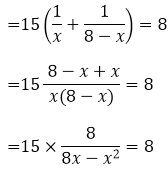⇒ 120 = 8(8x – x2)

⇒ 120 = 64x – 8x2

⇒ 8x– 64x + 120 = 0

⇒ 8(x– 8x + 15) = 0

⇒ x– 8x + 15 = 0

Solving for x by factorisation method, we have

⇒ x– 5x – 3x + 15 = 0

⇒ x(x – 5) – 3(x – 5) = 0

⇒ (x – 5)(x – 3) = 0

Now, either x – 5 = 0 ⇒ x = 5

Or, x – 3 = 0 ⇒ x = 3

Thus, the two numbers are 5 and 3, respectively.

10. The sum of a number and its positive square root is 6/25. Find the numbers.

Solution:

Let the number be x.

According to the question, we have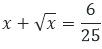Let us assume that x = y2,

So, we get

y2 + y = 6/25

⇒ 25y+ 25y – 6 = 0

⇒ 25y+ 30y – 5y – 6 = 0

⇒ 5y(5y + 6) – 1(5y + 6) = 0

⇒ (5y + 6) (5y – 1) = 0

Now, either 5y + 6 = 0 ⇒ y = -6/5

Or, 5y – 1 = 0 ⇒ y = 1/5

Since it’s given that only positive square root is to be considered, we take y = 1/5 only

Thus, x = (1/5)2 = 1/25

Hence, the number is 1/25.

11. The sum of a number and its square is 63/4, Find the numbers.

Solution:

Let the number be x.

So, its square will be x2.

From the question, it’s given that sum of the number, and its square is 63/4

Which means,

x + x2 = 63/4

⇒ 4x + 4x2 = 63

⇒ 4x2 + 4x – 63 = 0

Solving for x by factorisation method, we have

⇒ 4x2 + 18x – 14x – 63 = 0

⇒ 2x(2x + 9) – 7(2x – 9) = 0

⇒ (2x – 7)(2x + 9) = 0

Now, either 2x -7 = 0 ⇒ x = 7/2

Or, 2x + 9 = 0 ⇒ x = -9/2

Thus, the numbers are 7/2 and -9/2.

12. There are three consecutive integers such that the square of the first increased by the product of the other two gives 154. What are the integers?

Solution:

Let’s consider the three consecutive numbers to be x, x + 1, x + 2, respectively. And x is the first integer of the sequence.

From the question, it’s understood that

x+ (x + 1)(x + 2) = 154

⇒ x+ x+ 3x + 2 = 154

⇒ 2x+ 3x – 152 = 0

Solving for x by factorisation method, we have

⇒ 2x+ 19x – 16x – 152 = 0

⇒ x(2x + 19) – 8(2x – 19) = 0

⇒ (2x – 19)(x – 8) = 0

Now, either 2x – 19 = 0 ⇒ x = 19/2 (which is not an integer)

Or, x – 8 = 0 ⇒ x = 8

Hence, considering x = 8, the three consecutive integers are 8, 9 and 10.

13. The product of two successive integral multiples of 5 is 300. Determine the multiples.

Solution:

Given that the product of two successive integral multiples of 5 is 300

Let’s assume the integers be 5x and 5(x+1), where x and x+1 are two consecutive multiples

Then, according to the question, we have

5x[5(x + 1)] = 300

⇒ 25x(x + 1) = 300

⇒ x+ x = 12

⇒ x+ x – 12 = 0

Solving for x by factorisation method, we have

⇒ x+ 4x – 3x – 12 = 0

⇒ x(x + 4) – 3(x + 4) = 0

⇒ (x + 4)(x – 3) = 0

Now, either x + 4 = 0 ⇒ x = -4

Or, x – 3 = 0 ⇒ x = 3

For, x = – 4

5x = – 20 and 5(x + 1) = -15

And, for x = 3

5x = 15 and 5(x + 1) = 20

Thus, the two successive integral multiples can be 15, 20 or -15 and -20, respectively.

14. The sum of the squares of two numbers is 233, and one of the numbers is 3, less than twice the other number. Find the numbers.

Solution:

Let one of the numbers be x. Then the other number will be 2x – 3.

From the question,

x+ (2x – 3)2 = 233

⇒ x+ 4x+ 9 – 12x = 233

⇒ 5x– 12x – 224 = 0

⇒ 5x– 40x + 28x – 224 = 0

⇒ 5x(x – 8) + 28(x – 8) = 0

⇒ (5x + 28) (x – 8) = 0

Now, 5x + 28 cannot be 0

so, x – 8 = 0 ⇒ x = 8

Considering the value of x = 8, we have

2x – 3 = 2(8) – 3 = 16 – 3 = 13

Thus, the two numbers are 8 and 13, respectively.

15. Find the consecutive even integers whose squares have the sum 340.

Solution:

Let’s consider the three consecutive even numbers to be 2x and 2x + 2, respectively.

From the question, it’s given that sum of the squares of these integers is 340.

Which means,

(2x)2 + (2x + 2)2 = 340

⇒ 4x2 + 4x2 + 8x + 4 = 340

⇒ 8x2 + 8x – 336 = 0

⇒ 8(x2 + x – 42) = 0

⇒ x2 + x – 42 = 0

Solving for x by factorisation method, we have

⇒ x2 + 7x – 6x – 42 = 0

⇒ (x + 7)(x – 6) = 0

Thus, x can be either -7 or 6.

If x = -7, the number are -14 (2x(-7)) and -12 (2x(-7) + 2)

Similarly, if x = 6, the numbers are 12 and 14.

Therefore, the consecutive integers are either -14, -12 or 12, 14.

16. The difference of two numbers is 4. If the difference of their reciprocals is 4/21, find the numbers.

Solution:

Let the two numbers be x and x – 4, respectively.

It’s given that the difference of two numbers is 4.

Now, from the question, we have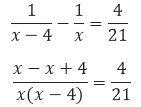⇒ 84 = 4x(x – 4)

⇒ x– 4x – 21 = 0

Solving for x by factorisation method, we have

⇒ x– 7x + 3x – 21 = 0

⇒ (x – 7)(x + 3) = 0

Now, either x – 7 = 0 ⇒ x = 7

Or, x + 3 = 0 ⇒ x = -3

Thus, the required numbers are – 3 and 7, respectively.

17. Find two natural numbers which differ by 3 and whose squares have the sum 117.

Solution:

Let the numbers be x and x – 3, as it’s given that the number differ by 3.

From the question, it’s given that sum of squares of these numbers is 117.

x+ (x – 3)= 117

⇒ x+ x+ 9 – 6x – 117 = 0

⇒ 2x– 6x – 108 = 0

⇒ x– 3x – 54 = 0

Solving for x by factorisation method, we have

⇒ x– 9x + 6x – 54 = 0

⇒ x(x – 9) + 6(x – 9) = 0

⇒ (x – 9)(x + 6) = 0

Now, either x – 9 = 0 ⇒ x = 9

Or, x + 6 = 0 ⇒ x = – 6

Considering only the positive value of x as natural numbers are always positive, i.e., x = 9.

So, x – 3 = 6.

Thus, the two numbers are 6 and 9, respectively.

18. The sum of the squares of three consecutive natural numbers is 149. Find the numbers.

Solution:

Let the three consecutive natural numbers be x, x + 1, and x + 2, respectively.

From the question, we have

x+ (x +1 )+ (x + 2)2 = 149

⇒ x+ x2 + x+ 1 + 2x + 4 + 4x = 149

⇒ 3x2 + 6x – 144 = 0

⇒ x+ 2x – 48 = 0 [dividing by 3 on both sides]

Solving for x by factorisation method, we have

x2  + 8x – 6x – 48 = 0

⇒ x(x + 8) – 6(x + 8) = 0

⇒ (x + 8)(x – 6) = 0

Now, either x + 8 = 0 ⇒ x = – 8

Or, x – 6 = 0 ⇒ x = 6

Considering only the positive value of x, which is 6, and discarding the negative value as the numbers considered are natural numbers.

So, x = 6, x + 1 = 7 and x + 2 = 8.

Thus, the three consecutive numbers are 6, 7, and 8, respectively.

19. The sum of two numbers is 16. The sum of their reciprocals is 1/3. Find the numbers.

Solution:

Let’s consider one of the two natural numbers as x, and then the other number will be 16 – x, as it’s given that their sum is 16.

Now, from the question, we can form the below equation.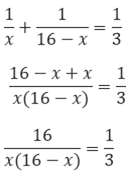⇒ 16x – x2 = 48

⇒ -16x + x+ 48 = 0

⇒ x– 16x + 48 = 0

Solving for x by factorisation method, we have

⇒ x– 12x – 4x + 48 = 0

⇒ x(x – 12) – 4(x – 12) = 0

⇒ (x – 12)(x – 4) = 0

So, either x – 12 = 0 ⇒ x = 12

Or, x – 4 = 0 ⇒ x = 4

Thus, the two numbers are 4 and 12, respectively.

20. Determine two consecutive multiples of 3 whose product is 270.

Solution:

Let the two consecutive multiples of 3 be 3x and 3x + 3

From the question, it’s given that

3x*(3x + 3) = 270

⇒ x(3x + 3) = 90 [Dividing by 3 on both sides]

⇒ 3x+ 3x = 90

⇒ 3x+ 3x – 90 = 0

⇒ x+ x – 30 = 0

Solving for x by factorisation method, we have

x+ 6x – 5x – 30 = 0

⇒ x(x + 6) – 5(x + 6) = 0

⇒ (x + 6)(x – 5) = 0

Now, either x + 6 = 0 ⇒ x = – 6

Or, x – 5 = 0 ⇒ x = 5

Considering the positive value of x, we have only

x = 5, so 3x = 15 and 3x + 3 = 18.

Thus, the two consecutive multiples of 3 are 15 and 18, respectively.

21. The sum of a number and its reciprocal is 17/4. Find the number.

Solution:

Let the number be x.

Then from the question, we have

x + 1/x = 17/4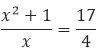⇒ 4(x2+1) = 17x

⇒ 4x+ 4 – 17x = 0

⇒ 4x+ 4 – 16x – x = 0

⇒ 4x(x – 4) – 1(x – 4) = 0

⇒ (4x – 1)(x – 4) = 0

Now, either x – 4 = 0 ⇒ x = 4

Or, 4x – 1 = 0 ⇒ x = 1/4

Thus, the value of x is 4.

### RD Sharma Solutions for Class 10 Maths Chapter 8 Exercise 8.8 Page No: 8.58

1. The speed of a boat in still water is 8km/hr. It can go 15 km upstream and 22 km downstream in 5 hours. Find the speed of the stream.

Solution:

Let the speed of the stream be x km/hr.

Given, the speed of the boat in still water is 8km/hr.

So, speed of downstream = (8 + x) km/hr

And, speed of upstream = (8 – x) km/hr

Using, speed = distance/ time

Time taken by the boat to go 15 km upstream = 15/(8 – x)hr

And, time taken by the boat to return 22 km downstream = 22/(8 + x)hr

From the question, the boat returns to the same point in 5 hr.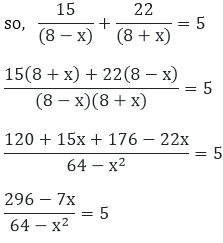5x– 7x + 296 – 320 = 0

5x2 – 7x – 24 = 0

5x2 – 15x + 8x – 24 = 0 [by factorisation method]

5x(x – 3) + 8(x – 3) = 0

(x – 3)(5x + 8) = 0

∴ x = 3, x = – 8/5

As the speed of the stream can never be negative, only the positive solution is considered.

Therefore, the speed of the stream is 3 km/hr.

2. A train, travelling at a uniform speed for 360 km, would have taken 48 minutes less to travel the same distance if its speed were 5 km/hr more. Find the original speed of the train.

Solution:

Let the original speed of the train be x km/hr.

When increased by 5, speed of the train = (x + 5) km/hr

Using, speed = distance/ time

Time taken by the train for the original uniform speed to cover 360 km = 360/x hr.

And, time taken by the train for increased speed to cover 360 km = 360/(x + 5) hr.

Given, that the difference in the times is 48 mins. ⇒ 48/60 hour

This can be expressed as below: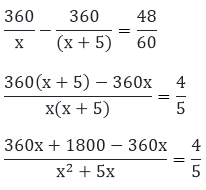1800(5) = 4(x2 + 5x)

9000 = 4x2 + 20x

4x+ 20x – 9000 = 0

x+ 5x – 2250 = 0

x2 + 50x – 45x – 2250 = 0 [by factorisation method]

x(x + 50) – 45(x + 50) = 0

(x + 50)(x – 45) = 0

∴ x = – 50 or x = 45

Since the speed of the train can never be negative, x = -50 is not considered.

Therefore, the original speed of the train is 45 km/hr.

3. A fast train takes one hour less than a slow train for a journey of 200 km. If the speed of the slow train is 10 km/hr less than that of the fast train, find the speed of the two trains.

Solution:

Let’s consider the speed of the fast train as x km/hr

Then, the speed of the slow train will be = (x -10) km/hr

Using, speed = distance/ time

Time taken by the fast train to cover 200 km = 200/x hr

And, time taken by the slow train to cover 200 km = 200/(x – 10) hr

Given, that the difference in the times is 1 hour.

This can be expressed as below: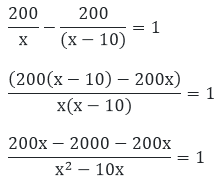x2– 10x = – 2000

x2 – 10x + 2000 = 0

x– 50x + 40x + 2000 = 0 [by factorisation method]

x(x – 50) + 40(x – 50) = 0

(x – 50)(x + 40) = 0

x = 50 or x = – 40

As the speed of a train can never be negative, we neglect x = -40

Thus, the speed of the fast train is 50 km/hr

And the speed of a slow train (50 – 10) = 40 km/hr

4. A passenger train takes one hour less for a journey of 150 km if its speed is increased 5 km/hr from its usual speed. Find the usual speed of the train.

Solution:

Let’s assume the usual speed of the train as x km/hr.

Then, the increased speed of the train = (x + 5) km/hr

Using, speed = distance/ time

Time taken by the train under usual speed to cover 150 km = 150/x hr

Time taken by the train under increased speed to cover 150 km = 150(x + 5)hr

Given, that the difference in the times is 1 hour.

This can be expressed as below: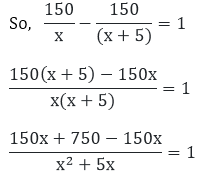750 = x2 + 5x

x2 + 5x – 750 = 0

x– 25x + 30x -750 = 0 [by factorisation method]

x(x – 25) + 30(x – 25) = 0

(x – 25) (x + 30) = 0

x = 25  or x = -30 (neglected as the speed of the train can never be negative)

Hence, the usual speed of the train is x = 25 km/hr

5. The time taken by a person to cover 150 km was 2.5 hrs more than the time taken in the return journey. If he returned at a speed of 10 km/hr more than the speed of going, what was the speed per hour in each direction?

Solution:

Let the ongoing speed of the person be x km/hr.

Then, the returning speed of the person is = (x + 10) km/hr (from the question)

Using, speed = distance/ time

Time taken by the person in going directly to cover 150 km = 150/x hr

And, time taken by the person in returning direction to cover 150 km = 150/(x + 10)hr

Given, that the difference in the times is 2.5 hour ⇒ 5/2 hours

This can be expressed as below: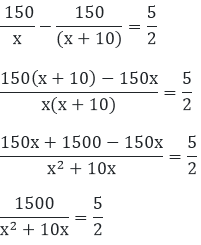3000 = 5x2 + 50x

5x2 + 50x – 3000 = 0

5(x2 + 10x – 600) = 0

x+ 10x – 600 = 0

x– 20x + 30x – 600 = 0 [by factorisation method]

x(x – 20) + 30(x – 20) = 0

(x – 20)(x + 30) = 0

x = 20  or x = -30(neglected) As the speed of train can never be negative.

Thus, x = 20 Then, (x + 10) (20 + 10) = 30

Therefore, the ongoing speed of the person is 20km/hr.

And the returning speed of the person is 30 km/hr.

6. A plane left 40 minutes late due to bad weather, and in order to reach the destination, 1600 km away in time, it had to increase its speed by 400 km/hr from its usual speed. Find the usual speed of the plane.

Solution:

Let’s assume the usual speed of the plane to be x km/hr,

Then the increased speed of the plane is = (x + 4000) km/hr

Using, speed = distance/ time

Time taken by the plane under usual speed to cover 1600 km = 1600/x hr

Time taken by the plane under increased speed to cover 1600 km = 1600/(x + 400) hr

Given, that the difference in the times is 40mins ⇒ 40/60 hours

This can be expressed as below: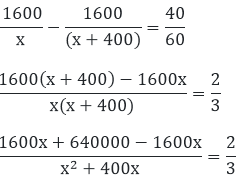1920000 = 2x2 + 800x

2x2 + 800x – 1920000 = 0

2(x2+ 400x – 960000) = 0

x2 + 400x – 960000 = 0

x2 – 800x + 1200x – 960000 = 0 [by factorisation method]

x(x – 800) + 1200(x – 800) = 0

(x – 800)(x + 1200) = 0

x = 800 or x = -1200 (neglected)

As the speed of the train can never be negative.

Thus, the usual speed of the train is 800 km/hr.

7. An aero plane takes 1 hour less for a journey of 1200 km if its speed is increased by 100 km/hr from its usual speed of the plane. Find its usual speed.

Solution:

Let’s consider the usual speed of the plane as x km/hr,

Then, the increased speed of the plane is = (x + 100) km/hr

Using, speed = distance/ time

Time taken by the plane under usual speed to cover 1200 km = 1200/x hr

Time taken by the plane under increased speed to cover 1200 km = 1200/(x + 100)hr

Given, that the difference in the times is 1 hour.

So, this can be expressed as below: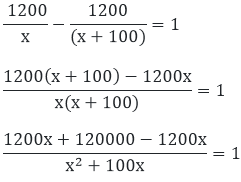120000 = x2 + 100x

x2 + 100x – 120000 = 0

x2 – 300x + 400x – 120000 = 0 [by factorisation method]

x(x – 300) + 400(x – 300) = 0

x = 300 or x = – 400 neglected as the speed of the aeroplane can never be negative.

Therefore, the usual speed of the train is 300 km/hr.

### RD Sharma Solutions for Class 10 Maths Chapter 8 Exercise 8.9 Page No: 8.61

1. Ashu is x years old, while his mother, Mrs. Veena, is x2 years old. Five years hence Mrs. Veena will be three times old as Ashu. Find their present ages.

Solution:

Given, Ashu’s present age is x years and his mother, Mrs. Veena, is x2 years.

After 5 years, Ashu’s age will be (x + 5) years

And his mother, Mrs. Veena’s age will be (x2 + 5) years

Given the relationship between their ages can be expressed as

x2 + 5 = 3(x + 5)

x2 + 5 = 3x + 15 x2 + 5 – 3x – 15 = 0

x2 – 5x + 2x + 10 = 0

x(x – 5) + 2(x – 5) = 0

(x – 5)(x + 2) = 0

x = 5 or x = – 2(neglected) since, the age can never be negative

Hence, Ashu’s present age is 5 years, and his mother’s age is 25 years.

2. The sum of the ages of a man and his son is 45 years. Five years ago, the product of their ages was four times the man’s age at the time. Find their present ages.

Solution:

Let the present age of the man be x years

Then, the present age of his son will be = (45 – x) years

Five years ago, man’s age = (x – 5) years

And, his son’s age = (45 – x – 5) = (40 – x) years

Given the relationship between their ages can be expressed as

(x – 5)(40 – x) = 4(x – 5)

40x – x2 + 5x – 200 = 4x – 20

-x2 + 45x – 200 = 4x – 20

-x2 + 45x – 200 – 4x + 20 = 0

– x2 + 41x – 180 = 0

x2 – 36x – 5x + 180 = 0 [By factorisation method]

x(x – 36) – 5(x – 36) = 0

(x – 36)(x – 5) = 0

x = 36 or  x = 5,

But, the father’s age can never be 5 years

Thus, when x = 36, 45 – x = 45 – 36 = 9

Therefore, the man’s present age is 36 years, and his son’s age is 9 years.

3. The product of Shikha’s age five years ago and her age 8 years later is 30, her age at both times being given in years. Find her present age.

Solution:

Let’s assume the present age of Shikha be x years

So, 8 years later, age of her = (x + 8) years

Five years ago, her age = (x – 5) years

Given the relationship between the ages can be expressed as

(x – 5)(x +8) = 30

x+ 8x – 5x – 40 = 30

x2 + 3x – 40 – 30 = 0

x+ 3x – 70 = 0 [By factorisation method]

x(x – 7) + 10(x – 7) = 0

(x – 7)(x + 10) = 0

x = 7 or x = -10 (neglected)

Age can never be negative.

Therefore, the present age of Shikha is 7 years.

4. The product of Ramu’s age (in years) five years ago and his age (in years) nine years later is 15. Determine Ramu’s present age.

Solution:

Let the present age of Ramu be x years

So, 9 years later, his age  = (x + 9) years

And, five years ago, his age = (x – 5) years

Given the relationship between the ages can be expressed as

(x – 5)(x + 5) = 15

x+ 9x – 5x – 45 = 15

x2 + 4x – 45 – 15 = 0

x2 + 4x – 60 = 0

x2 – 6x + 10x – 60 = 0 [By factorisation method]

x(x – 6) + 10(x – 6) = 0

(x – 6)(x + 10) = 0

x = 6  or  x = – 10(neglected) as the age can be never be negative.

Hence, the present age of Ramu is 6 years.

### RD Sharma Solutions for Class 10 Maths Chapter 8 Exercise 8.10 Page No: 8.64

1. The hypotenuse of a right triangle is 25 cm. The difference between the lengths of the other two sides of the triangle is 5 cm. Find the lengths of these sides.

Solution:

Let the length of one side of the right triangle be x cm

So, the other side will be = (x + 5) cm [as they differ by 5cm]

And given that hypotenuse = 25 cm

On applying Pythagoras’ Theorem, we have

x2 + (x + 5)2 = 252

x2 + x+ 10x + 25 = 625

2x+ 10x + 25 – 625 = 0

2x2 + 10x – 600 = 0

x2 + 5x – 300 = 0

x2 – 15x + 20x – 300 = 0 [By factorisation method]

x(x – 15) + 20(x -15) = 0

(x – 15)(x + 20) = 0

x = 15 or x = – 20 (neglected) As the side of the triangle can never be negative.

Thus, when x = 15 ⇒ x + 5 = 15 + 5 = 20

Hence, the length of the side of the right triangle is 15 cm, and other side is 20 cm.

2. The diagonal of a rectangular field is 60 meters more than the shorter side. If the longer side is 30 meters more than the shorter side, find the sides of the field.

Solution:

Let’s consider the length of the smaller side of the rectangle as x metres.

Then, the larger side will be (x + 30) metres and diagonal will be = (x + 60) metre

[From given relation]

Now, by using Pythagoras’ theorem, we have,

x2 + (x + 30)2 = (x + 60)2

x2 + x2 + 60x + 900 = x2 + 120x + 3600

2x2 + 60x + 900 – x2 – 120x – 3600 = 0

x2 – 60x – 2700 = 0

x2 – 90x + 30x – 2700 = 0 [By factorisation method]

x(x – 90) + 30(x – 90) = 0

(x – 90)(x + 30) = 0

x = 90   or x = -30 (this is neglected as the side of a rectangle can never be negative)

Therefore, we only take x = 90,

⇒ x + 30 = 90 + 30 = 120

Thus, the length of the smaller side of the rectangle is 90 metres, and the larger side is 120 metres.

### RD Sharma Solutions for Class 10 Maths Chapter 8 Exercise 8.11 Page No: 8.70

1. The perimeter of the rectangular field is 82 m, and its area is 400 m2. Find the breadth of the rectangle.

Solution: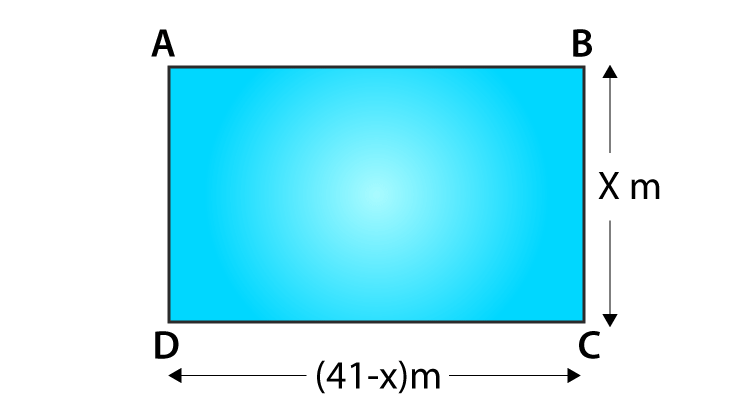Given,

Perimeter = 82 m and its area = 400 m2

Let the breadth of the rectangle be considered as x m.

We know that,

Perimeter of a rectangle = 2(length + breadth)

82 = 2(length + x)

41 = (length + x)

⇒Length = (41 – x)m

We also know that,

Area of the rectangle = length * breadth

400 = (41 – x)(x)

400 = 41x – x2

x– 41x + 400 = 0

x– 25x – 16x + 400 = 0

x(x – 25) – 16(x – 25) = 0

(x – 16)(x – 25) = 0

Now, either x – 16 = 0 ⇒ x = 16

Or, x – 25 = 0 ⇒ x = 25

Therefore, the breadth of the rectangle can be either 16 m or 25 m, respectively.

2. The length of the hall is 5 m more than its breadth. If the area of the floor of the hall is 84 m2, what are the length and breadth of the hall?

Solution: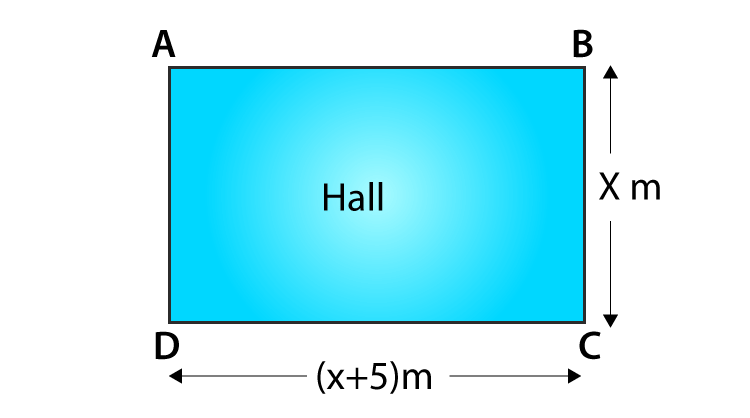Considering the breadth of the rectangle be x m

Then, the length of the hall is 5 m more than its breadth, i.e., = (x + 5) m

Given, the area of the hall is = 84 m2

As the shape of the hall is rectangular, its area is given by

Area of the rectangular hall = length * breadth

84 = x(x + 5)

x+ 5x – 84 = 0

x+ 12x – 7x – 84 = 0

x(x + 12) – 7(x + 12) = 0

(x + 12)(x – 7) = 0

Now, either x + 12 = 0 ⇒ x = – 12 (neglected since the side of a rectangle can never be negative)

Or, x – 7 = 0 ⇒ x = 7

So, only x = 7 is considered.

⇒ x + 5 = 12

Thus, the length and breadth of the rectangle are 7 and 12, respectively.

3. Two squares have sides x and (x + 4) cm. The sum of their area is 656 cm2. Find the sides of the square.

Solution:

Let S1 and S2 be the two squares.

And, let x cm be the side of square S1 and (x + 4) cm be the side of the square S2.

So,

Area of the square S1 = x2 cm2

Area of the square S2 =(x + 4)2 cm2

From the question, we have

Area of the square S+ Area of the square S= 656 cm2

⇒ x2 cm+ (x + 4)2 cm= 656 cm2

x2+ x+ 16 + 8x – 656 = 0

2x+ 16 + 8x – 656 = 0

2(x+ 4x – 320) = 0

x+4 x – 320 = 0

x+ 20x – 16x – 320 = 0

x(x + 20) – 16(x + 20) = 0

(x + 20)(x – 16) = 0

Now, either x + 20 = 0 ⇒ x = -20

Or, x – 16 = 0 ⇒ x = 16

As the value of x cannot be negative, we choose the value of x = 16 ⇒ x + 4 = 20

Therefore,

The side of the square S1= 16 cm

The side of the square S2 = 20 cm

4. The area of a right-angled triangle is 165 cm2. Determine its base and altitude if the latter exceeds the former by 7m.

Solution: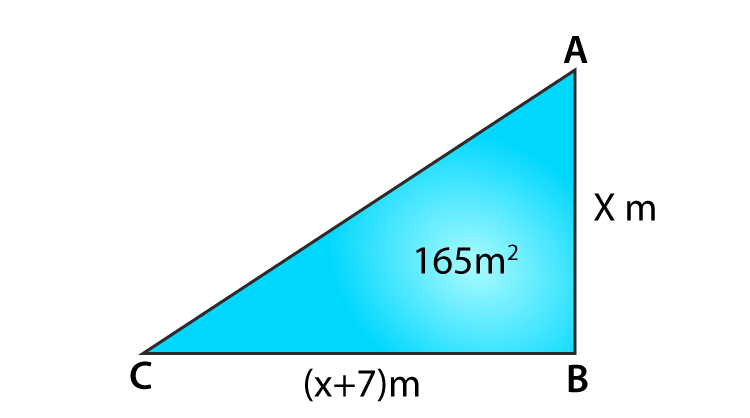Let the altitude of the right angle triangle be considered as x m.

So given that the altitude exceeds the base by 7m ⇒ altitude = (x – 7)m

We know that,

Area of the triangle = 1/2 × base × altitude

⇒ 165 = 1/2 × (x − 7) × x

x(x – 7) = 330

x– 7x – 330 = 0

x– 22x + 15x – 330 = 0

x(x – 22) + 15(x – 22) = 0

(x – 22)(x + 15) = 0

Now, either x – 22 = 0 ⇒ x = 22

Or, x + 15 = 0 ⇒ x = -15 (neglected)

Since the value of x cannot be negative, so the value of x = 22 is only considered

⇒ x – 7 = 15

Therefore the base and altitude of the right-angled triangle are 15 cm and 22 cm, respectively.

5. Is it possible to design a rectangular mango grove whose length is twice its breadth and the area is 800 m2? If so, find its length and breadth.

Solution: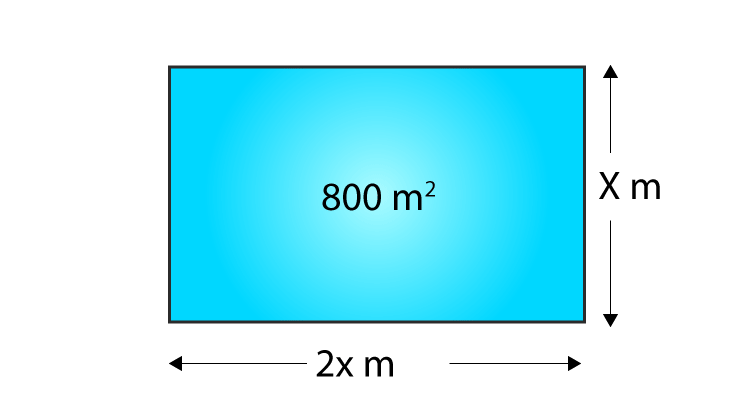Let the breadth of the rectangular mango grove be x m

Given that, the length of the rectangle is twice its breadth.

So, length = 2x

Area of the grove = 800 m2 (given)

We know that,

Area of the rectangle = length * breadth

800 = x(2x)

2x– 800 = 0

x– 400 = 0

⇒ x = √400 = 20 (neglecting the negative sq. root as the side can never be negative)

Therefore,

The breadth of the rectangular groove is 20 m.

And the length of the rectangular groove is 40 m.

Yes, it is possible to design a rectangular groove whose length is twice its breadth.

### RD Sharma Solutions for Class 10 Maths Chapter 8 Exercise 8.12 Page No: 8.73

1. A takes 10 days less than the time taken by B to finish a piece of work. If both A and B together can finish the work in 12 days, find the time taken by B to finish the work.

Solution:

Let’s consider that B takes x days to complete the piece of work.

So, B’s 1-day work = 1/x

Now, A takes 10 days less than that B to finish the same piece of work so that is (x –10) days.

⇒ A’s 1 day work = 1/(x – 10)

Same work both working together for 12 days, then

(A and B)’s 1 day’s work = 1/12

From the question, it’s understood that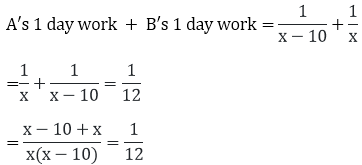⇒ 12(2x – 10) = x(x – 10)

⇒ 24x – 120 = x– 10x

⇒ x– 10x – 24x + 120 = 0

⇒ x– 34x + 120 = 0

⇒ x– 30x – 4x + 120 = 0

⇒ x(x – 30) – 4(x – 30) = 0

⇒ (x – 30)(x – 4) = 0

Now, either x – 30 = 0 ⇒ x = 30

Or, x – 4 = 0 ⇒ x = 4

It’s clear that the value of x cannot be less than 10, so the value of x = 30 is chosen.

Therefore, the time taken by B to finish the piece of work is 30 days.

2. If two pipes function simultaneously, a reservoir will be filled in 12 hours. One pipe fills the reservoir 10 hours faster than the other. How many hours will the second pipe take to fill the reservoir?

Solution:

Let’s consider that the faster pipe takes x hours to fill the reservoir.

Then, the portion of the reservoir filled by faster pipe in one hour = 1/x

Given that, the slower pipe takes 10 hours more than the faster pipe to fill the reservoir, which is (x + 10) hours.

Portion of reservoir filled by slower pipe = 1/(x + 10)

Now, it’s given that if both the pipes function simultaneously, the same reservoir can be filled in 12 hours

Thus, we know that the portion of the reservoir filled by both pipes in one hour = 1/12

Now,

Portion of reservoir filled by slower pipe in one hour + Portion of reservoir filled by faster pipe in one hour = 1/x + 1/(x + 10)

So, the portion of the reservoir filled by both pipes = 1/12, hence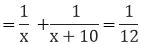⇒ 12(2x + 10) = x(x + 10)

⇒ x– 14x – 120 = 0

⇒ x– 20x + 6x – 120 = 0

⇒ x(x – 20) + 6(x – 20) = 0

⇒ (x – 20)(x + 6) = 0

Now, either x – 20 = 0 ⇒ x = 20

Or, x + 6 = 0 ⇒ x = – 6 (can be neglected)

Since the value of time cannot be negative.

Thus, the value of x is taken as 20 hours.

Therefore the time taken by the slower pipe to fill the reservoir = x + 10 = 30 hours.

### RD Sharma Solutions for Class 10 Maths Chapter 8 Exercise 8.13 Page No: 8.80

1. A piece of cloth costs Rs. 35. If the piece were 4 m longer and each metre costs Rs. 1 less, the cost would remain unchanged. How long is the piece?

Solution:

Let’s assume the length of the cloth to be ‘a’ metre.

Given, a piece of cloth costs Rs. 35, and if the piece were 4 m longer and each metre costs Rs. 1 less, the cost remains unchanged.

From the above condition, we have

Cost of 1m of cloth = 35/a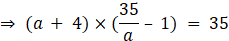⇒ (a + 4)(35 – a) = 35a

⇒ 35a + 140 – a2 – 4a = 35a

⇒ a2 + 4a – 140 = 0

Solving for ‘a’ by factorisation method,

⇒ a2 + 14a – 10a – 140 = 0

⇒ a(a + 14) – 10(a + 14) = 0

⇒ (a – 10)(a + 14) = 0

⇒ a = 10 m or a = -14 (neglected)

Since, the length of a cloth can never be negative.

So, a = 10

Hence, the piece of cloth was 10 m long.

2. Some students planned a picnic. The budget for food was Rs. 480. But eight of these failed to go and, thus, the cost of food for each member increased by Rs. 10. How many students attended the picnic?

Solution:

Let the number of students who planned the picnic be ‘x’.

And given, the budget for the food was Rs. 480

So, the cost of food for each member = 480/x

Also given, eight of these failed to go, and hence the cost of food for each member increased by Rs. 10

This can be expressed as below: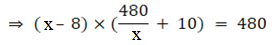⇒ (x – 8)(480 + 10x) = 480x

⇒ 480x + 10x2 – 3840 – 80x = 480x

⇒ x2 – 8x – 384 = 0

⇒ x2 – 24x + 16x – 384 = 0

⇒ x(x – 24) + 16(x – 24) = 0

⇒ (x + 16)(x – 24) = 0

⇒ x = 24 or

x = -16 (this solution is neglected since the number of students can never be negative.)

Hence, the number of students who planned the picnic was 24.

And, the number of students who attended the picnic = 24 – 8 = 16

3. A dealer sells an article for Rs. 24 and gains as much per cent as the cost price of the article. Find the cost price of the article.

Solution:

Let the cost price be assumed as Rs x.

Given, the dealer sells an article for Rs. 24 and gains as much per cent as the cost price of the article.

It’s given that he gains as much as the cost price of the article, thus, Gain% = x%

Gain% =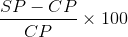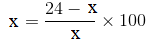⇒ x2 = (24-x)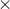100

⇒ x2 + 100x – 2400 = 0

⇒ x2 + 120x – 20x – 2400 = 0

⇒ (x + 120)(x – 20) = 0

⇒ x = 20 or -120

Since money cannot be negative, we call neglect -120

⇒ x = 20

Therefore, the cost price of the article is Rs 20

4. Out of a group of swans, 7/2 times the square root of the total number are playing on the share of a pond. The two remaining ones are swinging in the water. Find the total number of swans.

Solution:

Let’s assume the number of swans in the pond be ‘a’.

Given that, out of a group of swans, 7/2 times the square root of the total number are playing on the share of a pond. The two remaining ones are swinging in the water.

So, by expressing the total number of swans in the equation, we have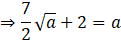⇒ 7√a = 2a – 4

On squaring both sides,

⇒ 49a = 4a2 + 16 – 16a

⇒ 4a2 – 65a + 16 = 0

⇒ 4a2 – 64a – a + 16 = 0 [By factorisation method]

⇒ 4a(a – 16) – (a – 16) = 0

⇒ (4a – 1)(a – 16) = 0

⇒ a =1/4 or a = 16

Since the number of swans can only be a natural number, we can neglect the solution of a = 1/4

Hence, the total number of swans is 16.

5. If the list price of a toy is reduced by Rs. 2, a person can buy 2 toys more for Rs. 360. Find the original price of the toy.

Solution:

Let the original price of the toy be ‘x’.

Given that, when the list price of a toy is reduced by Rs. 2, the person can buy 2 toys more for Rs. 360.

The number of toys he can buy at the original price of Rs. 360 = 360/x

According to the question,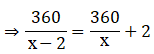⇒ 360x = (x – 2)(360 + 2x)

⇒ 360x = 360x + 2x2 – 720 – 4x

⇒ x2 – 2x – 360 = 0

⇒ x2 – 20x + 18x – 360 = 0 [By factorisation method]

⇒ x(x – 20) + 18(x – 20) = 0

⇒ (x + 18)(x – 20) = 0

⇒ x + 18 = 0 or x – 20 = 0

⇒ x = -18 or x = 20

As the price can’t be negative, a = -18 is neglected.

Thus, the original price of the toy is Rs 20.

6. Rs. 9000 was divided equally among a certain number of persons. Had there been 20 more persons, each would have got Rs. 160 less. Find the original number of persons.

Solution:

Let’s consider the original number of people as ‘a’.

Given that, Rs. 9000 were divided equally among a certain number of persons. Had there been 20 more persons, each would have got Rs. 160 less.

The amount which each receives when persons are present = 9000/a

Expressing the given condition we have,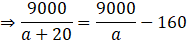⇒ 9000a = (9000 – 160a)(a + 20)

⇒ 9000a = 9000a + 180000 – 160a2 – 3200a

⇒ a2 + 20a – 1125 = 0

⇒ a2 + 45a – 25a – 1125 = 0

⇒ a(a + 45) – 25(a + 45) = 0

⇒ (a – 25)(a + 45) = 0

⇒ a = 25 or a = -45 (neglected as the number of people can never be negative)

Therefore, the original number of people = 25.

## Frequently Asked Questions on RD Sharma Solutions for Class 10 Maths Chapter 8

Q1

### How many exercises are there in RD Sharma Solutions for Class 10 Maths Chapter 8?

The 8th Chapter of RD Sharma Solutions for Class 10 Maths has 13 exercises. The first exercise deals with the topic of determination of quadratic equations, the second exercise has questions of finding formulation of quadratic equations, the third exercise deals with finding the various ways of finding their zeros or roots like factorisation, completing the square, and by using the quadratic formula. The fourth and remaining exercises have questions based on applications of quadratic equations in daily life situations. By solving these exercises, students are able to answer all the questions based on quadratic equations and their properties.
Q2

### Is BYJU’S website providing answers for RD Sharma Solutions for Class 10 Maths Chapter 8 Quadratic Equations?

Yes, you can avail the PDFs of RD Sharma Solutions for Class 10 Maths Quadratic Equations at BYJU’S. These solutions are formulated by expert faculty at BYJU’S in a unique way. Also, we provide solutions for Classes 1 to 12 RD Sharma Textbooks in free PDFs. Students who wish to score high in exams are advised to solve the RD Sharma Textbook.
Q3

### Mention the important concepts that you learn in RD Sharma Solutions for Class 10 Maths Chapter 8 Quadratic Equations.

The concepts presented in RD Sharma Solutions for Class 10 Maths Chapter 8 Quadratic Equations are as follows: